# Using the 'BayesFactor' package, version 0.9.2+

## Richard D. Morey

Stable version: CRAN page - Package NEWS (including version changes)

Development version: Development page - Development package NEWS

### Introduction

The BayesFactor package enables the computation of Bayes factors in standard designs, such as one- and two- sample designs, ANOVA designs, and regression. The Bayes factors are based on work spread across several papers. This document is designed to show users how to compute Bayes factors using the package by example. It is not designed to present the models used in the comparisons in detail; for that, see the BayesFactor help and especially the references listed in this manual. Complete references are given at the end of this document.

If you need help or think you've found a bug, please use the links at the top of this document to contact the developers. When asking a question or reporting a bug, please send example code and data, the exact errors you're seeing (a cut-and-paste from the R console will work) and instructions for reproducing it. Also, report the output of BFInfo() and sessionInfo(), and let us know what operating system you're running.

The BayesFactor package must be installed and loaded before it can be used. Installing the package can be done in several ways and will not be covered here. Once it is installed, use the library function to load it:

library(BayesFactor)


This command will make the BayesFactor package ready to use.

### Some useful functions

The table below lists some of the functions in the BayesFactor package that will be demonstrated in this manual. For more complete help on the use of these functions, see the corresponding help() page in R.

Function Description
ttestBF Bayes factors for one- and two- sample designs
anovaBF Bayes factors comparing many ANOVA models
regressionBF Bayes factors comparing many linear regression models
generalTestBF Bayes factors for all restrictions on a full model (0.9.4+)
lmBF Bayes factors for specific linear models (ANOVA or regression)
correlationBF Bayes factors for linear correlations
proportionBF Bayes factors for tests of single proportions
contingencyTableBF Bayes factors for contingency tables
posterior Sample from the posterior distribution of the numerator of a Bayes factor object
recompute Recompute a Bayes factor or MCMC chain, possibly increasing the precision of the estimate
compare Compare two models; typically used to compare two models in BayesFactor MCMC objects

#### Functions to manipulate Bayes factor objects

The t test section below has examples showing how to manipulate Bayes factor objects, but all these functions will work with Bayes factors generated from any function in the BayesFactor package.

Function Description
/ Divide two Bayes factor objects to create new model comparisons, or invert with 1/
t “Flip” (transpose) a Bayes factor object
c Concatenate two Bayes factor objects together, assuming they have the same denominator
[ Use indexing to select a subset of the Bayes factors
plot plot a Bayes factor object
sort Sort a Bayes factor object
is.na Determine whether a Bayes factor object contains missing values
head,tail Return the n highest or lowest Bayes factor in an object
max, min Return the highest or lowest Bayes factor in an object
which.max,which.min Return the index of the highest or lowest Bayes factor
as.vector Convert to a simple vector (denominator will be lost!)
as.data.frame Convert to data.frame (denominator will be lost!)

### One- and two-sample designs (t tests)

The ttestBF function is used to obtain Bayes factors corresponding to tests of a single sample's mean, or tests that two independent samples have the same mean.

#### One-sample tests (and paired)

We use the sleep data set in R to demonstrate a one-sample t test. This is a paired design; for details about the data set, see ?sleep. One way of analyzing these data is to compute difference scores by subtracting a participant's score in one condition from their score in the other:

data(sleep)

## Compute difference scores
diffScores = sleep$extra[1:10] - sleep$extra[11:20]

## Traditional two-tailed t test
t.test(diffScores)

##
##  One Sample t-test
##
## data:  diffScores
## t = -4, df = 9, p-value = 0.003
## alternative hypothesis: true mean is not equal to 0
## 95 percent confidence interval:
##  -2.46 -0.70
## sample estimates:
## mean of x
##     -1.58


We can do a Bayesian version of this analysis using the ttestBF function, which performs the “JZS” t test described by Rouder, Speckman, Sun, Morey, and Iverson (2009). In this model, the true standardized difference $$\delta=(\mu-\mu_0)/\sigma_\epsilon$$ is assumed to be 0 under the null hypothesis, and $$\text{Cauchy}(\text{scale}=r)$$ under the alternative. The default $$r$$ scale in BayesFactor for t tests is $$\sqrt{2}/2$$. See ?ttestBF for more details.

bf = ttestBF(x = diffScores)
## Equivalently:
## bf = ttestBF(x = sleep$extra[1:10],y=sleep$extra[11:20], paired=TRUE)
bf

## Bayes factor analysis
## --------------
##  Alt., r=0.707 : 17.3 ±0%
##
## Against denominator:
##   Null, mu = 0
## ---
## Bayes factor type: BFoneSample, JZS


The bf object contains the Bayes factor, and shows the numerator and denominator models for the Bayes factor comparison. In our case, the Bayes factor for the comparison of the alternative versus the null is 17.259. After the Bayes factor is a proportional error estimate on the Bayes factor.

There are a number of operations we can perform on our Bayes factor, such as taking the reciprocal:

1 / bf

## Bayes factor analysis
## --------------
##  Null, mu=0 : 0.0579 ±0%
##
## Against denominator:
##   Alternative, r = 0.707106781186548, mu =/= 0
## ---
## Bayes factor type: BFoneSample, JZS


or sampling from the posterior of the numerator model:

chains = posterior(bf, iterations = 1000)
summary(chains)

##
## Iterations = 1:1000
## Thinning interval = 1
## Number of chains = 1
## Sample size per chain = 1000
##
## 1. Empirical mean and standard deviation for each variable,
##    plus standard error of the mean:
##
##        Mean     SD Naive SE Time-series SE
## mu    -1.42  0.436   0.0138         0.0154
## sig2   2.02  1.157   0.0366         0.0395
## delta -1.11  0.427   0.0135         0.0162
## g      6.26 58.623   1.8538         1.8538
##
## 2. Quantiles for each variable:
##
##         2.5%    25%   50%    75%  97.5%
## mu    -2.289 -1.705 -1.43 -1.141 -0.597
## sig2   0.744  1.270  1.69  2.446  5.223
## delta -1.973 -1.383 -1.08 -0.813 -0.347
## g      0.176  0.592  1.13  2.928 33.734


The posterior function returns a object of type BFmcmc, which inherits the methods of the mcmc class from the coda package. We can thus use summary, plot, and other useful methods on the result of posterior. If we were unhappy with the number of iterations we sampled for chains, we can recompute with more iterations, and then plot the results:

chains2 = recompute(chains, iterations = 10000)
plot(chains2[,1:2])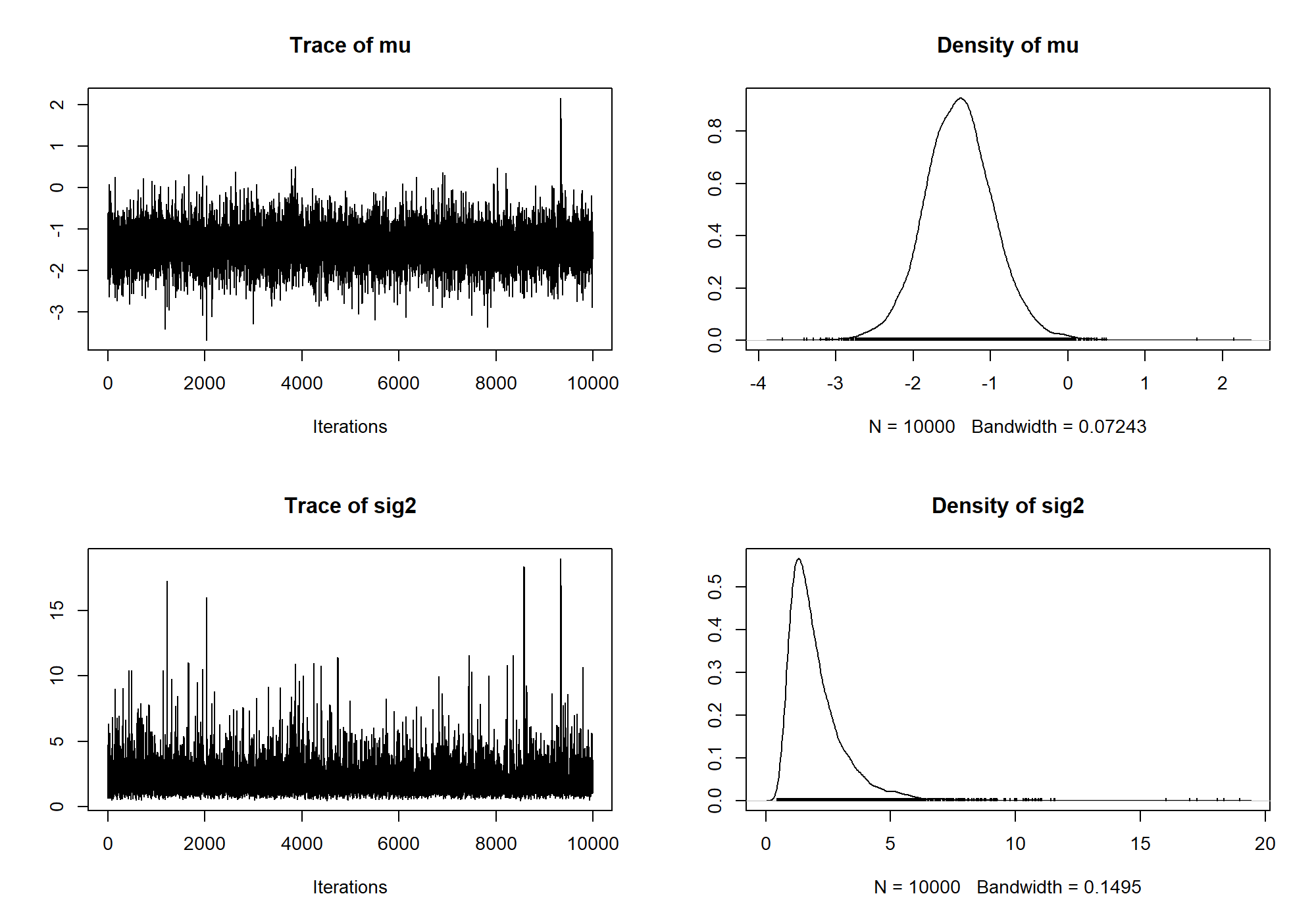Directional hypotheses can also be tested with ttestBF (Morey & Rouder, 2011). The argument nullInterval can be passed as a vector of length 2, and defines an interval to compare to the point null. If null interval is defined, two Bayes factors are returned: the Bayes factor of the null interval against the alternative, and the Bayes factor of the complement of the interval to the point null.

Suppose, for instance, we wanted to test the one-sided hypotheses that $$\delta<0$$ versus the point null. We set nullInterval to c(-Inf,0):

bfInterval = ttestBF(x = diffScores, nullInterval=c(-Inf,0))
bfInterval

## Bayes factor analysis
## --------------
##  Alt., r=0.707 -Inf<d<0    : 34.4  ±0%
##  Alt., r=0.707 !(-Inf<d<0) : 0.101 ±0%
##
## Against denominator:
##   Null, mu = 0
## ---
## Bayes factor type: BFoneSample, JZS


We may not be interested in tests against the point null. If we are interested in the Bayes factor test that $$\delta<0$$ versus $$\delta>0$$ we can compute it using the result above. Since the object contains two Bayes factors, both with the same denominator, and $\left.\frac{A}{C}\middle/\frac{B}{C}\right. = \frac{A}{B},$ we can divide the two Bayes factors in bfInferval to obtain the desired test:

bfInterval / bfInterval

## Bayes factor analysis
## --------------
##  Alt., r=0.707 -Inf<d<0 : 341 ±0%
##
## Against denominator:
##   Alternative, r = 0.707106781186548, mu =/= 0 !(-Inf<d<0)
## ---
## Bayes factor type: BFoneSample, JZS


The Bayes factor is about 340.

When we have multiple Bayes factors that all have the same denominator, we can concatenate them into one object using the c function. Since bf and bfInterval both share the point null denominator, we can do this:

allbf = c(bf, bfInterval)
allbf

## Bayes factor analysis
## --------------
##  Alt., r=0.707             : 17.3  ±0%
##  Alt., r=0.707 -Inf<d<0    : 34.4  ±0%
##  Alt., r=0.707 !(-Inf<d<0) : 0.101 ±0%
##
## Against denominator:
##   Null, mu = 0
## ---
## Bayes factor type: BFoneSample, JZS


The object allbf now contains three Bayes factors, all of which share the same denominator. If you try to concatenate Bayes factors that do not share the same denominator, BayesFactor will return an error.

When you have a Bayes factor object with several numerators, there are several interesting ways to manipulate them. For instance, we can plot the Bayes factor object to obtain a graphical representation of the Bayes factors:

plot(allbf)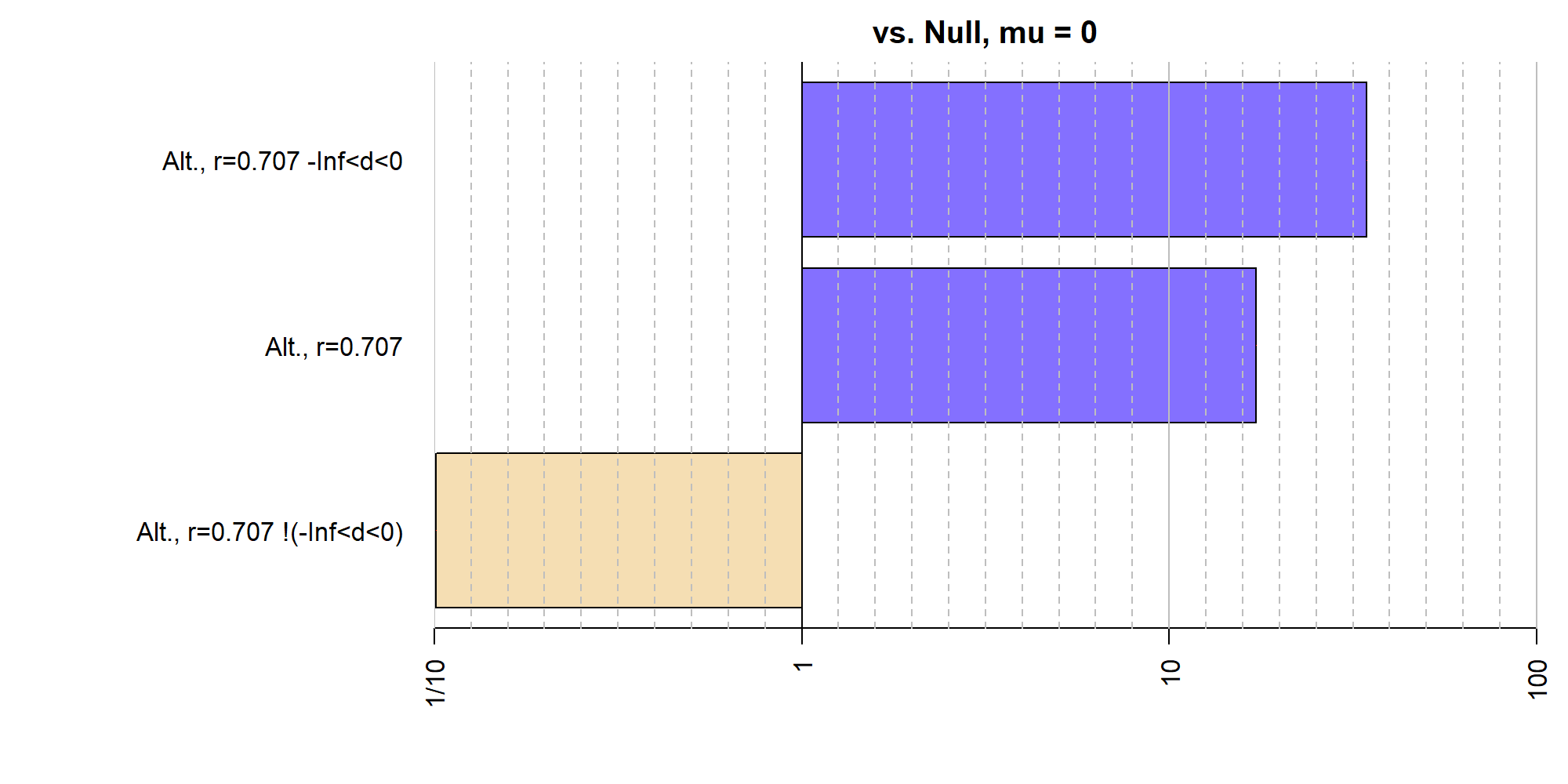We can also divide a Bayes factor object by itself — or by a subset of itself — to obtain pairwise comparisons:

bfmat = allbf / allbf
bfmat

##                            denominator
## numerator                   Alt., r=0.707 Alt., r=0.707 -Inf<d<0
##   Alt., r=0.707                   1.00000                0.50146
##   Alt., r=0.707 -Inf<d<0          1.99416                1.00000
##   Alt., r=0.707 !(-Inf<d<0)       0.00584                0.00293
##                            denominator
## numerator                   Alt., r=0.707 !(-Inf<d<0)
##   Alt., r=0.707                                   171
##   Alt., r=0.707 -Inf<d<0                          341
##   Alt., r=0.707 !(-Inf<d<0)                         1


The resulting object is of type BFBayesFactorList, and is a list of Bayes factor comparisons all of the same numerators compared to different denominators. The resulting matrix can be subsetted to return individual Bayes factor objects, or new BFBayesFactorLists:

bfmat[,2]

## Bayes factor analysis
## --------------
##  Alt., r=0.707             : 0.501   ±0%
##  Alt., r=0.707 -Inf<d<0    : 1       ±0%
##  Alt., r=0.707 !(-Inf<d<0) : 0.00293 ±0%
##
## Against denominator:
##   Alternative, r = 0.707106781186548, mu =/= 0 -Inf<d<0
## ---
## Bayes factor type: BFoneSample, JZS

bfmat[1,]

##                denominator
## numerator       Alt., r=0.707 Alt., r=0.707 -Inf<d<0 Alt., r=0.707 !(-Inf<d<0)
##   Alt., r=0.707             1                  0.501                       171


and they can also be transposed:

bfmat[,1:2]

##                            denominator
## numerator                   Alt., r=0.707 Alt., r=0.707 -Inf<d<0
##   Alt., r=0.707                   1.00000                0.50146
##   Alt., r=0.707 -Inf<d<0          1.99416                1.00000
##   Alt., r=0.707 !(-Inf<d<0)       0.00584                0.00293

t(bfmat[,1:2])

##                         denominator
## numerator                Alt., r=0.707 Alt., r=0.707 -Inf<d<0
##   Alt., r=0.707                   1.00                  0.501
##   Alt., r=0.707 -Inf<d<0          1.99                  1.000
##                         denominator
## numerator                Alt., r=0.707 !(-Inf<d<0)
##   Alt., r=0.707                                171
##   Alt., r=0.707 -Inf<d<0                       341


If these values are desired in matrix form, the as.matrix function can be used to obtain a matrix.

#### Two-sample test (independent groups)

The ttestBF function can also be used to compute Bayes factors in the two sample case as well. We use the chickwts data set to demonstrate the two-sample t test. The chickwts data set has six groups, but we reduce it to two for the demonstration.

data(chickwts)

## Restrict to two groups
chickwts = chickwts[chickwts$feed %in% c("horsebean","linseed"),] ## Drop unused factor levels chickwts$feed = factor(chickwts$feed) ## Plot data plot(weight ~ feed, data = chickwts, main = "Chick weights")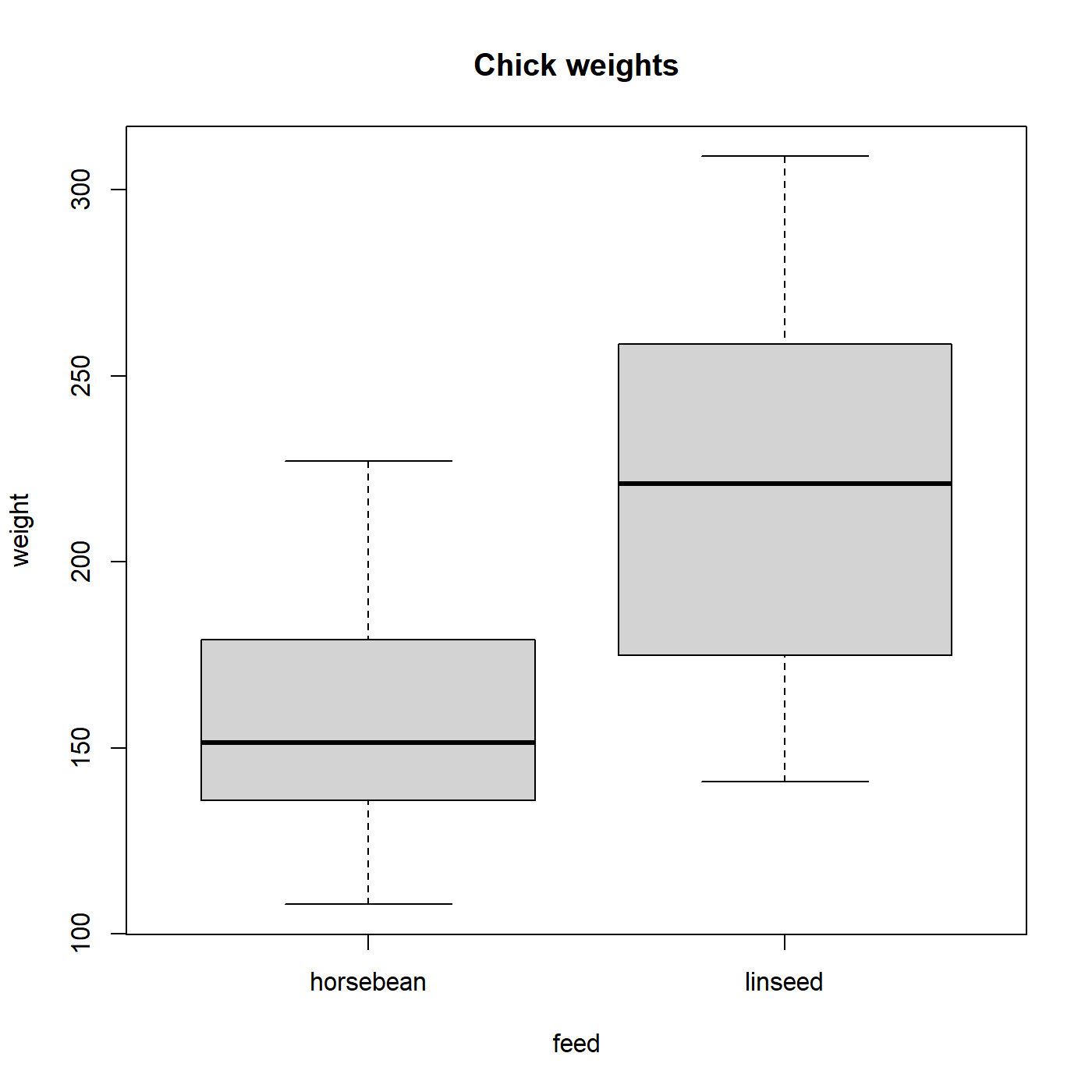Chick weight appears to be affected by the feed type. ## traditional t test t.test(weight ~ feed, data = chickwts, var.eq=TRUE)  ## ## Two Sample t-test ## ## data: weight by feed ## t = -3, df = 20, p-value = 0.008 ## alternative hypothesis: true difference in means between group horsebean and group linseed is not equal to 0 ## 95 percent confidence interval: ## -100.2 -16.9 ## sample estimates: ## mean in group horsebean mean in group linseed ## 160 219  We can also compute the corresponding Bayes factor. There are two ways of specifying a two-sample test: the formula interface and through the x and y arguments. We show the formula interface here: ## Compute Bayes factor bf = ttestBF(formula = weight ~ feed, data = chickwts) bf  ## Bayes factor analysis ## -------------- ##  Alt., r=0.707 : 5.98 ±0% ## ## Against denominator: ## Null, mu1-mu2 = 0 ## --- ## Bayes factor type: BFindepSample, JZS  As before, we can sample from the posterior distribution for the numerator model: chains = posterior(bf, iterations = 10000) plot(chains[,2])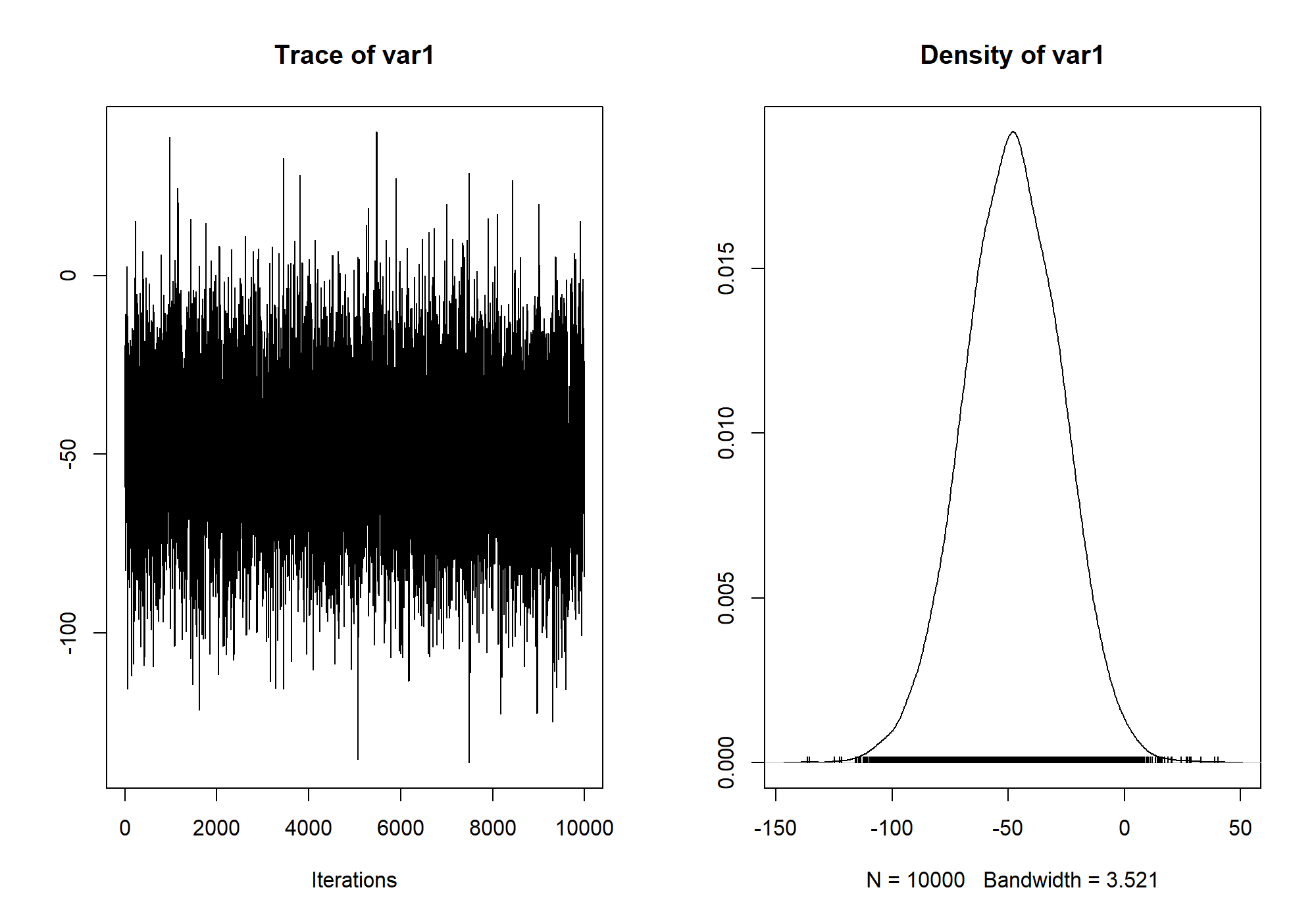Note that the samples assume an (equivalent) ANOVA model; see ?ttestBF and for notes on the differences in interpretation of the $$r$$ scale parameter between the two models. ### Meta-analytic t tests (0.9.8+) Rouder and Morey (2011; link) discuss a meta-analytic extension of the $$t$$ test, whereby multiple $$t$$ statistics, along with their corresponding sample sizes, are combined in a single meta-analytic analysis. The $$t$$ statistics are assumed to arise from a a common effect size $$\delta$$. The prior for the effect size $$\delta$$ is the same as that for the $$t$$ tests described above. The meta.ttestBF function is used to perform meta-analytic $$t$$ tests. It requires as input a vector of $$t$$ statistics, and one or two vectors of sample sizes (arguments n1 and n2). For a set of one-sample $$t$$ statistics, n1 should be provided; for two-sample analyses, both n1 and n2 should be provided. As an example, we will replicate the analysis of Rouder & Morey (2011), using $$t$$ statistics from Bem (2010; see Rouder & Morey for reference). We begin by defining the one-sample $$t$$ statistics and sample sizes: ## Bem's t statistics from four selected experiments t = c(-.15, 2.39, 2.42, 2.43) N = c(100, 150, 97, 99)  Rouder and Morey opted for a one-sided analysis, and used an $$r$$ scale parameter of 1 (instead of the current default in BayesFactor of $$\sqrt{2}/2$$). bf = meta.ttestBF(t=t, n1=N, nullInterval=c(0,Inf), rscale=1) bf  ## Bayes factor analysis ## -------------- ##  Alt., r=1 0<d<Inf : 38.7 ±0% ##  Alt., r=1 !(0<d<Inf) : 0.00803 ±0% ## ## Against denominator: ## Null, d = 0 ## --- ## Bayes factor type: BFmetat, JZS  Notice that as above, the analysis yields a Bayes factor for our selected interval against the null, as well as the Bayes factor for the complement of the interval against the null. We can also sample from the posterior distribution of the standardized effect size $$\delta$$, as above, using the posterior function: ## Do analysis again, without nullInterval restriction bf = meta.ttestBF(t=t, n1=N, rscale=1) ## Obtain posterior samples chains = posterior(bf, iterations = 10000)  ## Independent-candidate M-H acceptance rate: 98%  plot(chains)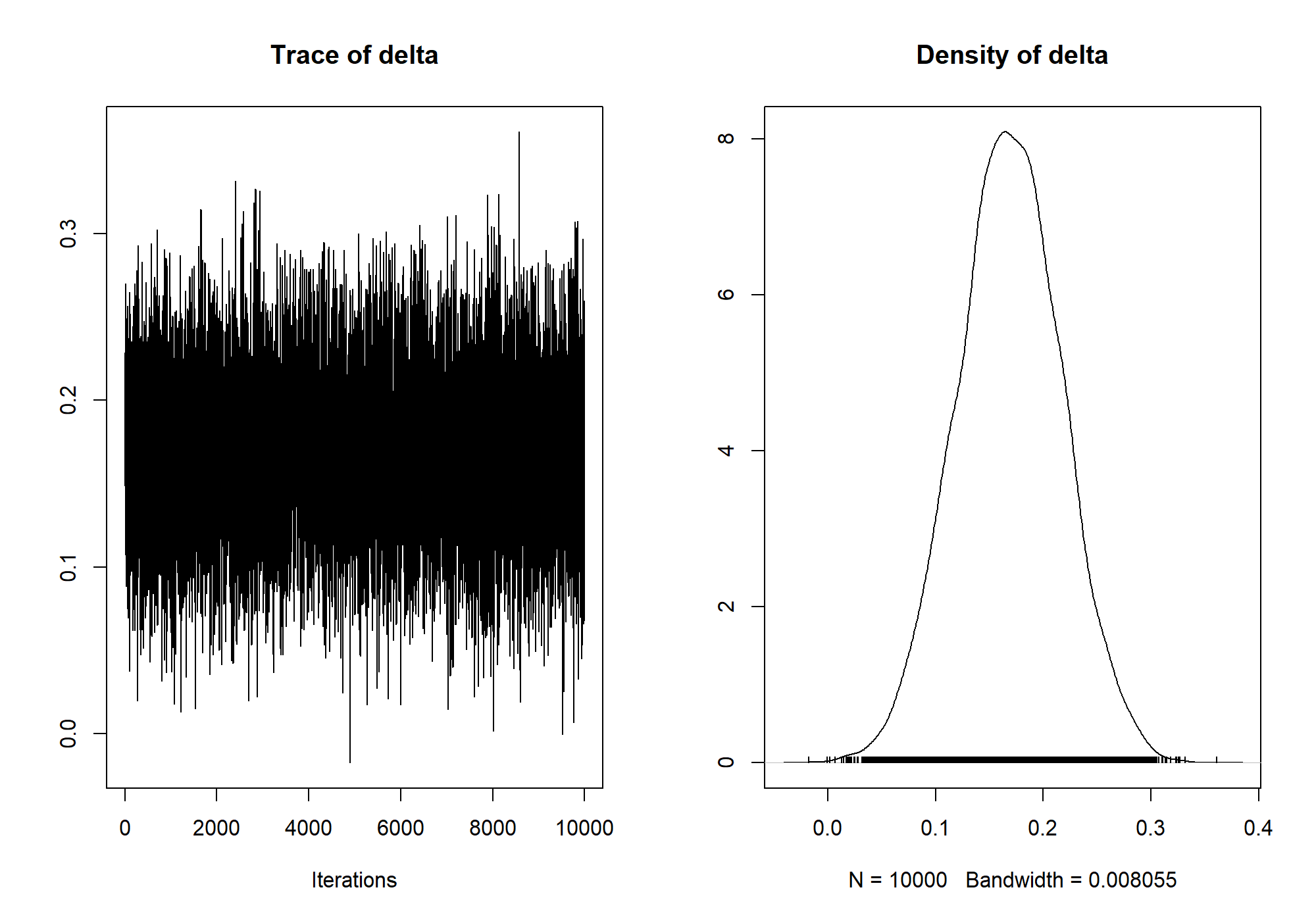Notice that the posterior samples will respect the nullInterval argument if given; in order to get unrestricted samples, perform an analysis with no interval restriction and pass it to the posterior function. See ?meta.ttestBF for more information. ### ANOVA The BayesFactor package has two main functions that allow the comparison of models with factors as predictors (ANOVA): anovaBF, which computes several model estimates at once, and lmBF, which computes one comparison at a time. We begin by demonstrating a 3x2 fixed-effect ANOVA using the ToothGrowth data set. For details about the data set, see ?ToothGrowth. #### Fixed-effects ANOVA The ToothGrowth data set contains three columns: len, the dependent variable, each of which is the length of a guinea pig's tooth after treatment with Vitamin C; supp, which is the supplement type (orange juice or ascorbic acid); and dose, which is the amount of Vitamin C administered. data(ToothGrowth) ## Example plot from ?ToothGrowth coplot(len ~ dose | supp, data = ToothGrowth, panel = panel.smooth, xlab = "ToothGrowth data: length vs dose, given type of supplement")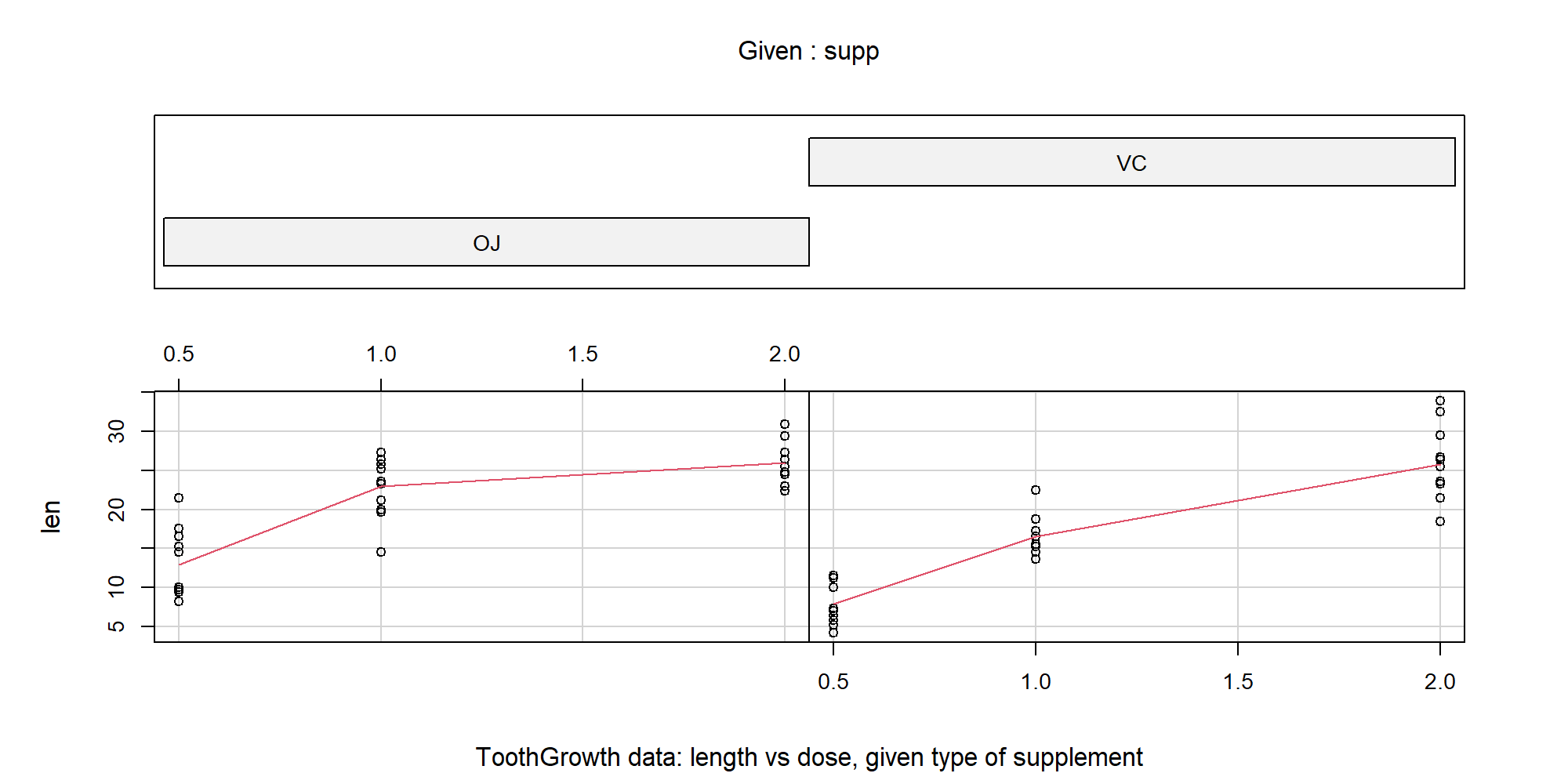## Treat dose as a factor ToothGrowth$dose = factor(ToothGrowth$dose) levels(ToothGrowth$dose) = c("Low", "Medium", "High")

summary(aov(len ~ supp*dose, data=ToothGrowth))

##             Df Sum Sq Mean Sq F value  Pr(>F)
## supp         1    205     205   15.57 0.00023 ***
## dose         2   2426    1213   92.00 < 2e-16 ***
## supp:dose    2    108      54    4.11 0.02186 *
## Residuals   54    712      13
## ---
## Signif. codes:  0 '***' 0.001 '**' 0.01 '*' 0.05 '.' 0.1 ' ' 1


There appears to be a large effect of the dosage, a small effect of the supplement type, and perhaps a hint of an interaction. The anovaBF function will compute the Bayes factors of all models against the intercept-only model; by default, it will choose the subset of all models in which which an interaction can only be included if all constituent effects or interactions are included (argument whichModels is set to withmain, indicating that interactions can only enter in with their main effects). However, this setting can be changed, as we will demonstrate. First, we show the default behavior.

bf = anovaBF(len ~ supp*dose, data=ToothGrowth)
bf

## Bayes factor analysis
## --------------
##  supp                    : 1.2      ±0.01%
##  dose                    : 4.98e+12 ±0%
##  supp + dose             : 2.92e+14 ±1.58%
##  supp + dose + supp:dose : 7.44e+14 ±1.01%
##
## Against denominator:
##   Intercept only
## ---
## Bayes factor type: BFlinearModel, JZS


The function will build the requested models from the terms included in the right-hand side of the formula; we could have specified the sum of the two terms, and we would have gotten the same models.

The Bayes factor analysis is consistent with the classical ANOVA analysis; the favored model is the full model, with both main effects and the two-way interaction. Suppose we were interested in comparing the two main-effects model and the full model to the dose-only model. We could use indexing and division, along with the plot function, to see a graphical representation of these comparisons:

plot(bf[3:4] / bf)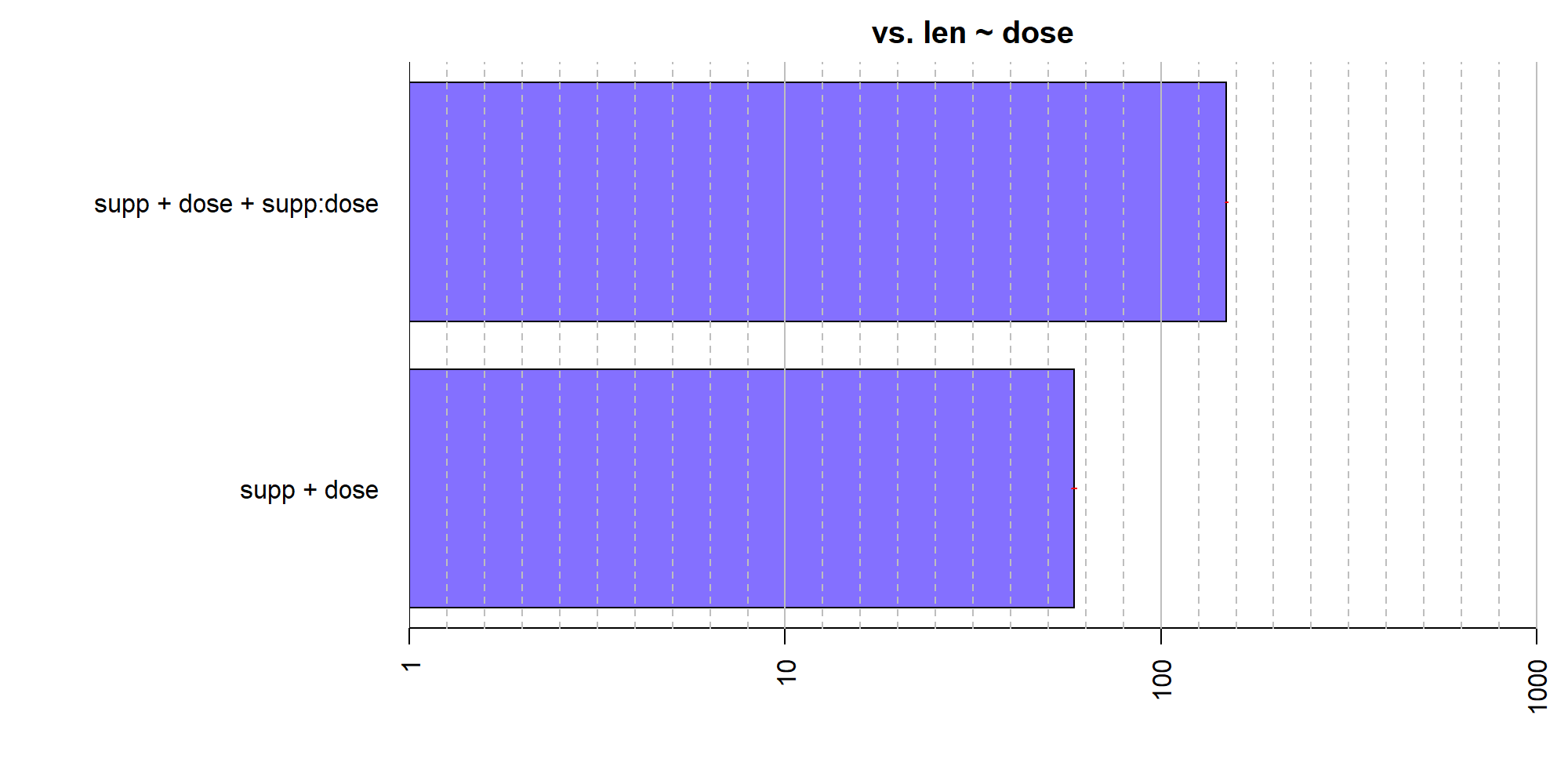The model with the main effect of supp and the supp:dose interaction is preferred quite strongly over the dose-only model.

There are a number of other options for how to select subsets of models to test. The whichModels argument to anovaBF controls which subsets are tested. As described previously, the default is withmain, where interactions are only allowed if all constituent sub-effects are included. The other three options currently available are all, which tests all models; top, which includes the full model and all models that can be formed by removing one interaction or main effect; and bottom, which adds single effects one at a time to the null model.

The argument whichModels='all' should be used with caution: a three-way ANOVA model will contain $$2^{2^3-1}-1 = 127$$ model comparisons; a four-way ANOVA, $$2^{2^4-1}-1 = 32767$$ models, and a five-way ANOVA just over 2.1 billion models. Depending on the speed of your computer, a four-way ANOVA may take several hours to a day, but a five-way ANOVA is probably not feasible.

One alternative is whichModels='top', which reduces the number of comparisons to $$2^k-1$$, where $$k$$ is the number of factors, which is manageable. In orthogonal designs, one can construct tests of each main effect or interaction by comparing the full model to the model with all effects except the one of interest:

bf = anovaBF(len ~ supp*dose, data=ToothGrowth, whichModels="top")
bf

## Bayes factor top-down analysis
## --------------
## When effect is omitted from supp + dose + supp:dose , BF is...
##  Omit dose:supp : 0.385    ±3.32%
##  Omit dose      : 7.11e-16 ±12.2%
##  Omit supp      : 0.011    ±4.17%
##
## Against denominator:
##   len ~ supp + dose + supp:dose
## ---
## Bayes factor type: BFlinearModel, JZS


Note that all of the Bayes factors are less than 1, indicating that removing any effect from the full model is deleterious.

Another way we can reduce the number of models tested is simply to test only specific models of interest. In the example above, for instance, we might want to compare the model with the interaction to the model with only the main effects, if our effect of interest was the interaction. We can do this with the lmBF function.

bfMainEffects = lmBF(len ~ supp + dose, data = ToothGrowth)
bfInteraction = lmBF(len ~ supp + dose + supp:dose, data = ToothGrowth)
## Compare the two models
bf = bfInteraction / bfMainEffects
bf

## Bayes factor analysis
## --------------
##  supp + dose + supp:dose : 2.79 ±2.51%
##
## Against denominator:
##   len ~ supp + dose
## ---
## Bayes factor type: BFlinearModel, JZS


The model with the interaction effect is preferred by a factor of about 3.

Suppose that we were unhappy with the ~2.5% proportional error on the Bayes factor bf. anovaBF and lmBF use Monte Carlo integration to estimate the Bayes factors. The default number of Monte Carlo samples is 10,000 but this can be increased. We could use the recompute to reduce the error. The recompute function performs the sampling required to build the Bayes factor object again:

newbf = recompute(bf, iterations = 500000)
newbf

## Bayes factor analysis
## --------------
##  supp + dose + supp:dose : 2.74 ±0.51%
##
## Against denominator:
##   len ~ supp + dose
## ---
## Bayes factor type: BFlinearModel, JZS


The proportional error is now below 1%.

As before, we can use MCMC methods to estimate parameters through the posterior function:

## Sample from the posterior of the full model
chains = posterior(bfInteraction, iterations = 10000)
## 1:13 are the only "interesting" parameters
summary(chains[,1:13])

##
## Iterations = 1:10000
## Thinning interval = 1
## Number of chains = 1
## Sample size per chain = 10000
##
## 1. Empirical mean and standard deviation for each variable,
##    plus standard error of the mean:
##
##                         Mean    SD Naive SE Time-series SE
## mu                    18.819 0.487  0.00487        0.00487
## supp-OJ                1.679 0.488  0.00488        0.00549
## supp-VC               -1.679 0.488  0.00488        0.00549
## dose-Low              -8.069 0.683  0.00683        0.00705
## dose-Medium            0.910 0.680  0.00680        0.00666
## dose-High              7.159 0.684  0.00684        0.00710
## supp:dose-OJ.&.Low     0.562 0.603  0.00603        0.00616
## supp:dose-OJ.&.Medium  0.822 0.621  0.00621        0.00723
## supp:dose-OJ.&.High   -1.384 0.663  0.00663        0.00833
## supp:dose-VC.&.Low    -0.562 0.603  0.00603        0.00616
## supp:dose-VC.&.Medium -0.822 0.621  0.00621        0.00723
## supp:dose-VC.&.High    1.384 0.663  0.00663        0.00833
## sig2                  14.039 2.772  0.02772        0.03260
##
## 2. Quantiles for each variable:
##
##                         2.5%    25%    50%    75%  97.5%
## mu                    17.880 18.492 18.817 19.142 19.765
## supp-OJ                0.719  1.356  1.679  2.002  2.647
## supp-VC               -2.647 -2.002 -1.679 -1.356 -0.719
## dose-Low              -9.421 -8.516 -8.073 -7.608 -6.746
## dose-Medium           -0.418  0.457  0.904  1.370  2.234
## dose-High              5.808  6.696  7.167  7.615  8.516
## supp:dose-OJ.&.Low    -0.613  0.160  0.552  0.945  1.766
## supp:dose-OJ.&.Medium -0.354  0.404  0.801  1.223  2.093
## supp:dose-OJ.&.High   -2.740 -1.825 -1.365 -0.920 -0.154
## supp:dose-VC.&.Low    -1.766 -0.945 -0.552 -0.160  0.613
## supp:dose-VC.&.Medium -2.093 -1.223 -0.801 -0.404  0.354
## supp:dose-VC.&.High    0.154  0.920  1.365  1.825  2.740
## sig2                   9.625 12.062 13.692 15.615 20.373


And we can plot the posteriors of some selected effects:

plot(chains[,4:6])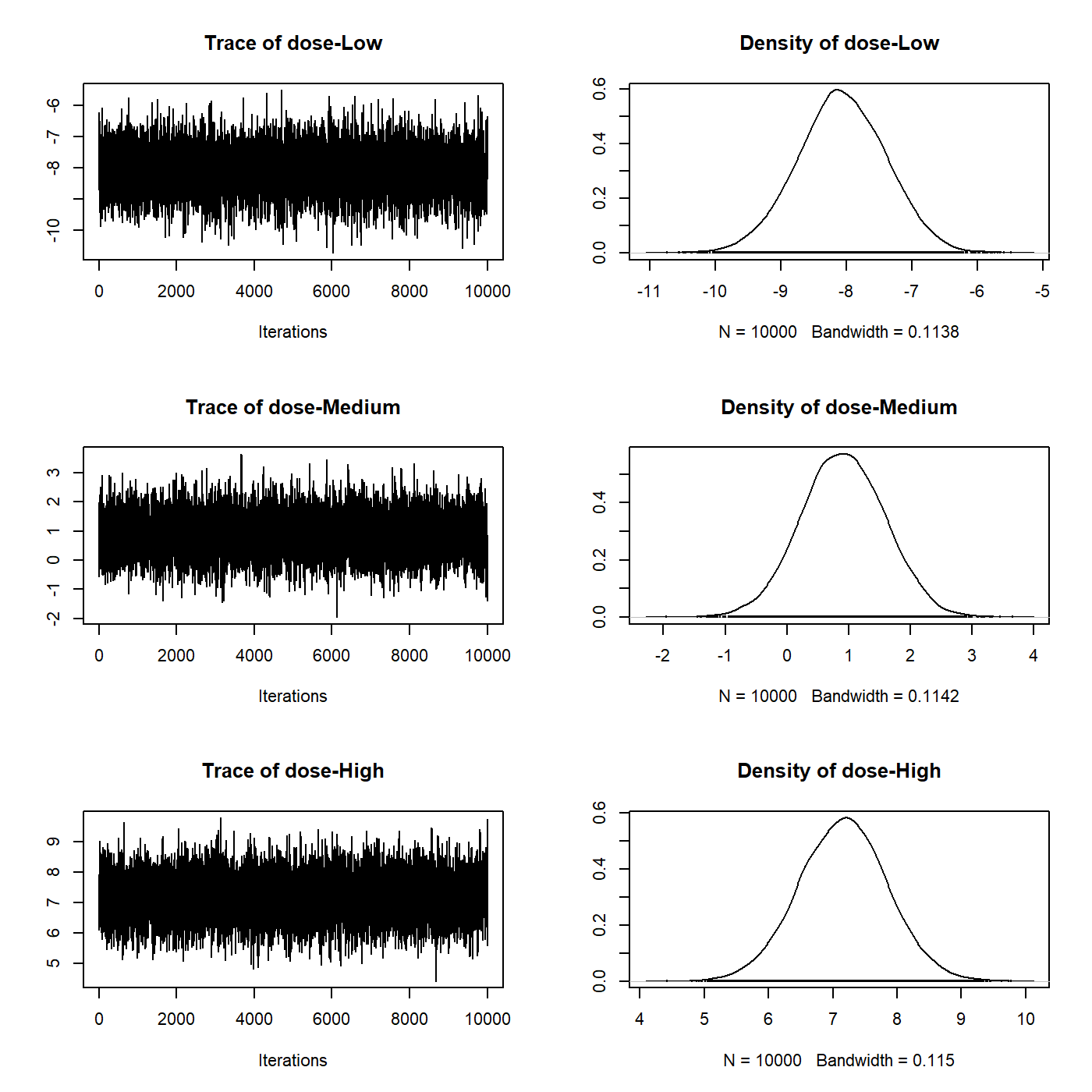#### Mixed models (including repeated measures)

In order to demonstrate the analysis of mixed models using BayesFactor, we will load the puzzles data set, which is part of the BayesFactor package. See ?puzzles for details. The data set consists of four columns: RT the dependent variable, which is the number of seconds that it took to complete a puzzle; ID which is a participant identifier; and shape and color, which are two factors that describe the type of puzzle solved. shape and color each have two levels, and each of 12 participants completed puzzles within combination of shape and color. The design is thus 2x2 factorial within-subjects.

We first load the data, then perform a traditional within-subjects ANOVA.

data(puzzles)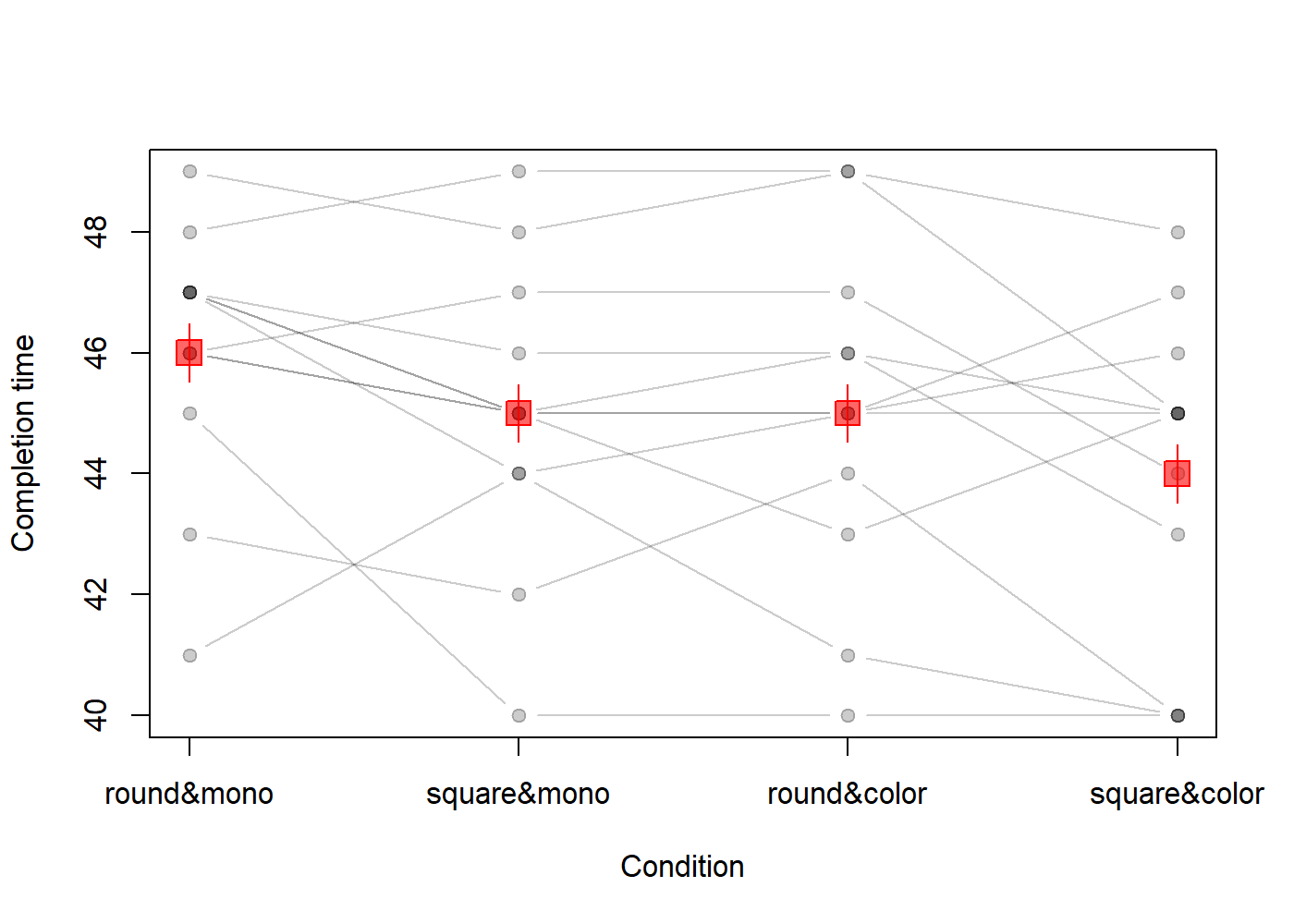(Code for plot omitted) Individual circles joined by lines show participants; red squares/lines show the means and within-subject standard errors. From the plot, there appear to be main effects of color and shape, but no interaction.

summary(aov(RT ~ shape*color + Error(ID/(shape*color)), data=puzzles))

##
## Error: ID
##           Df Sum Sq Mean Sq F value Pr(>F)
## Residuals 11    226    20.6
##
## Error: ID:shape
##           Df Sum Sq Mean Sq F value Pr(>F)
## shape      1   12.0   12.00    7.54  0.019 *
## Residuals 11   17.5    1.59
## ---
## Signif. codes:  0 '***' 0.001 '**' 0.01 '*' 0.05 '.' 0.1 ' ' 1
##
## Error: ID:color
##           Df Sum Sq Mean Sq F value Pr(>F)
## color      1   12.0   12.00    13.9 0.0033 **
## Residuals 11    9.5    0.86
## ---
## Signif. codes:  0 '***' 0.001 '**' 0.01 '*' 0.05 '.' 0.1 ' ' 1
##
## Error: ID:shape:color
##             Df Sum Sq Mean Sq F value Pr(>F)
## shape:color  1    0.0    0.00       0      1
## Residuals   11   30.5    2.77


The classical ANOVA appears to corroborate the impression from the plot. In order to compute the Bayes factor, we must tell anovaBF that ID is an additive effect on top of the other effects (as is typically assumed) and is a random factor. The anovaBF call below shows how this is done:

bf = anovaBF(RT ~ shape*color + ID, data = puzzles,
whichRandom="ID")


We alert anovaBF to the random factor using the whichRandom argument. whichRandom should contain a character vector with the names of all random factors in it. All other factors are assumed to be fixed. The anovaBF will find all the fixed effects in the formula, and compute the Bayes factor for the subset of combinations determined by the whichModels argument (see the previous section). Note that anovaBF does not test random factors; they are assumed to be nuisance factors. The null model in a test with random factors is not the intercept-only model; it is the model containing the random effects. The Bayes factor object bf thus now contains Bayes factors comparing various combinations of the fixed effects and an additive effect of ID against a denominator containing only ID:

bf

## Bayes factor analysis
## --------------
##  shape + ID                       : 2.81 ±0.91%
##  color + ID                       : 2.81 ±0.83%
##  shape + color + ID               : 11.9 ±3%
##  shape + color + shape:color + ID : 4.23 ±2.2%
##
## Against denominator:
##   RT ~ ID
## ---
## Bayes factor type: BFlinearModel, JZS


The main effects model is preferred against all models. We can plot the Bayes factor object to obtain a graphical representation of the model comparisons:

plot(bf)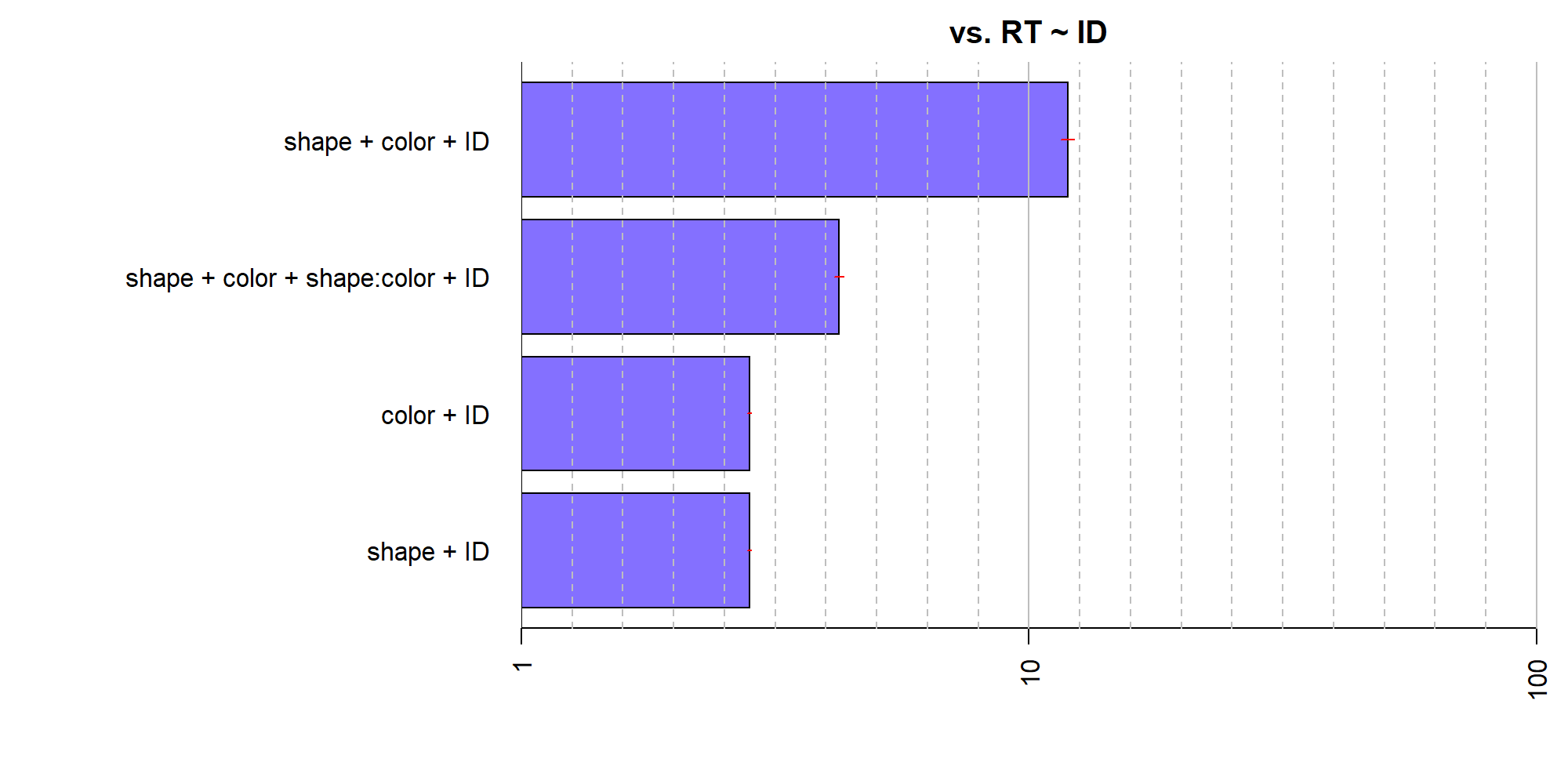Because the anovaBF function does not test random factors, we must use lmBF to build such tests. Doing so is straightforward. Suppose that we wished to test the random effect ID in the puzzles example. We might compare the full model shape + color + shape:color + ID to the same model without ID:

bfWithoutID = lmBF(RT ~ shape*color, data = puzzles)
bfWithoutID

## Bayes factor analysis
## --------------
##  shape * color : 0.143 ±1.14%
##
## Against denominator:
##   Intercept only
## ---
## Bayes factor type: BFlinearModel, JZS


But notice that the denominator model is the intercept-only model; the denominator in the previous analysis was the ID only model. We need to compare the model with no ID effect to the model with only ID:

bfOnlyID = lmBF(RT ~ ID, whichRandom="ID",data = puzzles)
bf2 = bfWithoutID / bfOnlyID
bf2

## Bayes factor analysis
## --------------
##  shape * color : 1.28e-06 ±1.14%
##
## Against denominator:
##   RT ~ ID
## ---
## Bayes factor type: BFlinearModel, JZS


Since our bf object and bf2 object now have the same denominator, we can concatenate them into one Bayes factor object:

bfall = c(bf,bf2)


and we can compare them by dividing:

bf / bf2

## Bayes factor analysis
## --------------
##  shape + color + shape:color + ID : 3307085 ±2.48%
##
## Against denominator:
##   RT ~ shape * color
## ---
## Bayes factor type: BFlinearModel, JZS


The model with ID is preferred by a factor of over 1 million, which is not surprising.

Any model that is a combination of fixed and random factors, including interations between fixed and random factors, can be constructed and tested with lmBF. anovaBF is designed to be a convenience function as is therefore somewhat limited in flexibility with respect to the models types it can test; however, because random effects are often nuisance effects, we believe anovaBF will be sufficient for most researchers' use.

### Linear regression

Model comparison in multiple linear regression using BayesFactor is done via the approach of Liang, Paulo, Molina, Clyde, and Berger (2008). Further discussion can be found in Rouder & Morey (in press). To demonstrate Bayes factor model comparison in a linear regression context, we use the attitude data set in R. See ?attitude. The attitude consists of the dependent variable rating, along with 6 predictors. We can use BayesFactor to compute the Bayes factors for many models simultaneously, or single Bayes factors against the model containing no predictors.

data(attitude)

## Traditional multiple regression analysis
lmObj = lm(rating ~ ., data = attitude)
summary(lmObj)

##
## Call:
## lm(formula = rating ~ ., data = attitude)
##
## Residuals:
##     Min      1Q  Median      3Q     Max
## -10.942  -4.356   0.316   5.543  11.599
##
## Coefficients:
##             Estimate Std. Error t value Pr(>|t|)
## (Intercept)  10.7871    11.5893    0.93   0.3616
## complaints    0.6132     0.1610    3.81   0.0009 ***
## privileges   -0.0731     0.1357   -0.54   0.5956
## learning      0.3203     0.1685    1.90   0.0699 .
## raises        0.0817     0.2215    0.37   0.7155
## critical      0.0384     0.1470    0.26   0.7963
## advance      -0.2171     0.1782   -1.22   0.2356
## ---
## Signif. codes:  0 '***' 0.001 '**' 0.01 '*' 0.05 '.' 0.1 ' ' 1
##
## Residual standard error: 7.07 on 23 degrees of freedom
## Multiple R-squared:  0.733,  Adjusted R-squared:  0.663
## F-statistic: 10.5 on 6 and 23 DF,  p-value: 1.24e-05


The period (.) is shorthand for all remaining columns, besides rating. The predictors complaints and learning appear most stongly related to the dependent variable, especially complaints. In order to compute the Bayes factors for many model comparisons at onces, we use the regressionBF function. The most obvious set of all model comparisons is all possible additive models, which is returned by default:

bf = regressionBF(rating ~ ., data = attitude)
length(bf)

##  63


The object bf now contains $$2^p-1$$, or 63, model comparisons. Large numbers of comparisons can get unweildy, so we can use the functions built into R to manipulate the Bayes factor object.

## Choose a specific model
bf["privileges + learning + raises + critical + advance"]

## Bayes factor analysis
## --------------
##  privileges + learning + raises + critical + advance : 51 ±0%
##
## Against denominator:
##   Intercept only
## ---
## Bayes factor type: BFlinearModel, JZS

## Best 6 models

## Bayes factor analysis
## --------------
##  complaints                      : 417939 ±0.01%
##  complaints + learning           : 207272 ±0%
##  complaints + learning + advance : 88042  ±0%
##  complaints + raises             : 77499  ±0%
##  complaints + privileges         : 75015  ±0%
##  complaints + advance            : 72760  ±0%
##
## Against denominator:
##   Intercept only
## ---
## Bayes factor type: BFlinearModel, JZS

## Worst 4 models
tail(bf, n=4)

## Bayes factor analysis
## --------------
##  privileges + critical + advance : 0.645 ±0%
##  critical                        : 0.449 ±0%
##  advance                         : 0.447 ±0%
##  critical + advance              : 0.239 ±0.01%
##
## Against denominator:
##   Intercept only
## ---
## Bayes factor type: BFlinearModel, JZS

## which model index is the best?
which.max(bf)

## complaints
##          1

## Compare the 5 best models to the best
bf2 = head(bf) / max(bf)
bf2

## Bayes factor analysis
## --------------
##  complaints                      : 1     ±0%
##  complaints + learning           : 0.496 ±0.01%
##  complaints + learning + advance : 0.211 ±0.01%
##  complaints + raises             : 0.185 ±0.01%
##  complaints + privileges         : 0.179 ±0.01%
##  complaints + advance            : 0.174 ±0.01%
##
## Against denominator:
##   rating ~ complaints
## ---
## Bayes factor type: BFlinearModel, JZS

plot(bf2)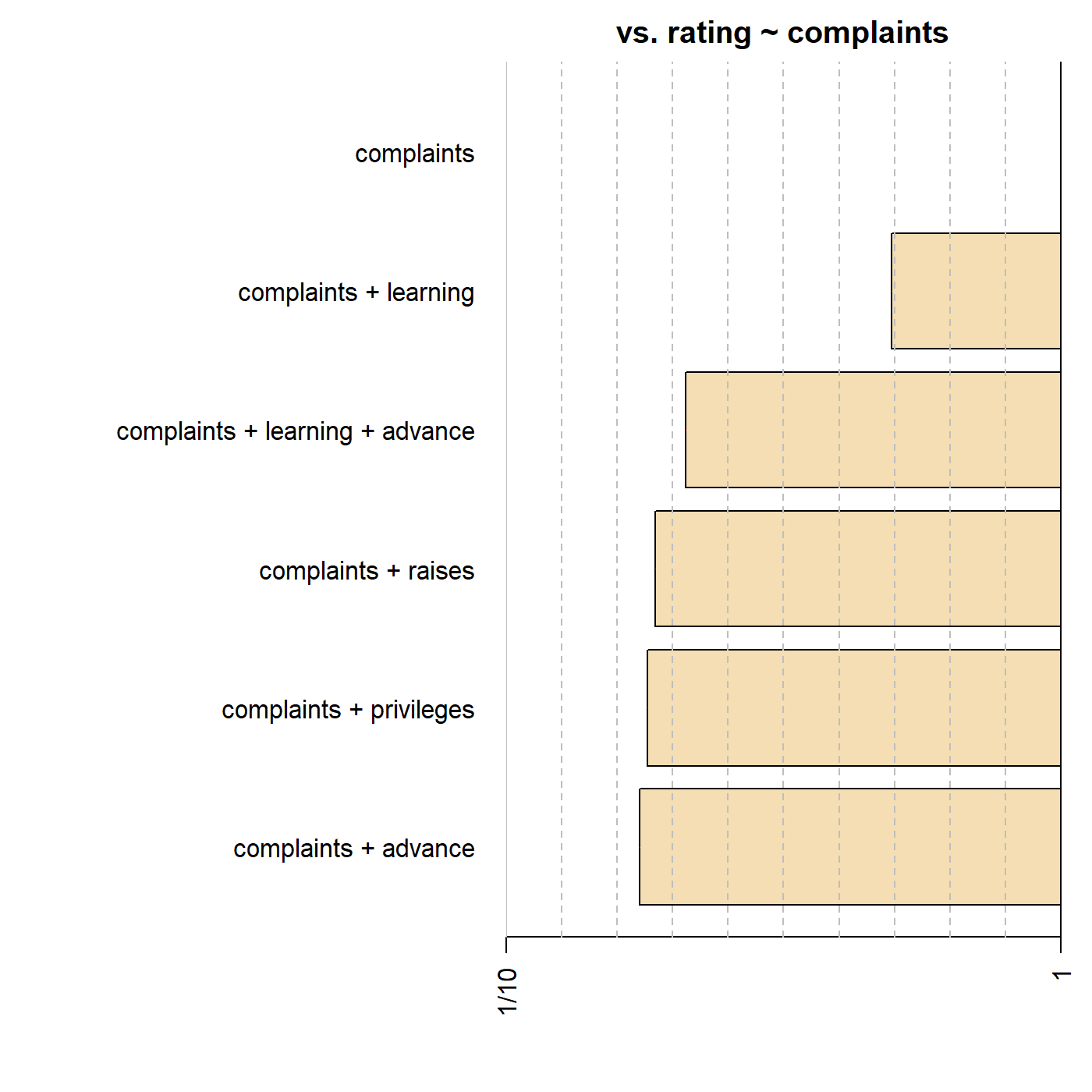The model preferred by Bayes factor is the complaints-only model, followed by the complaints + learning model, as might have been expected by the classical analysis.

We might also be interested in comparing the most complex model to all models that can be formed by removing a single covariate, or, similarly, comparing the intercept-only model to all models that can be formed by added a covariate. These comparisons can be done by setting the whichModels argument to 'top' and 'bottom', respectively. For example, for testing against the most complex model:

bf = regressionBF(rating ~ ., data = attitude, whichModels = "top")
## The seventh model is the most complex
bf

## Bayes factor top-down analysis
## --------------
## When effect is omitted from complaints + privileges + learning + raises + critical + advance , BF is...
##  Omit advance    : 1.73   ±0%
##  Omit critical   : 3.23   ±0%
##  Omit raises     : 3.13   ±0%
##  Omit learning   : 0.727  ±0%
##  Omit privileges : 2.92   ±0%
##  Omit complaints : 0.0231 ±0%
##
## Against denominator:
##   rating ~ complaints + privileges + learning + raises + critical + advance
## ---
## Bayes factor type: BFlinearModel, JZS

plot(bf)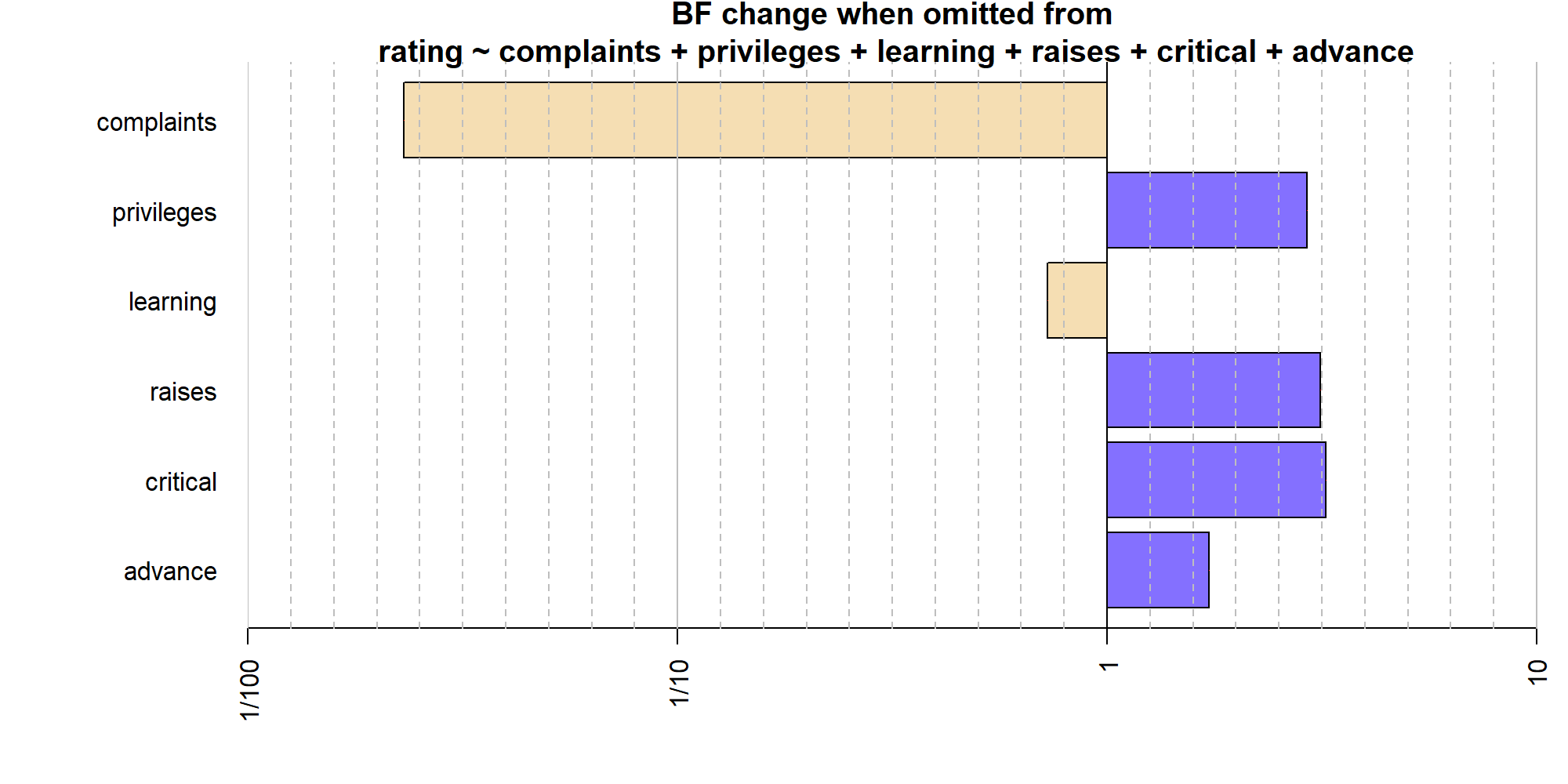With all other covariates in the model, the model containing complaints is preferred to the model not containing complaints by a factor of almost 80. The model containing learning, is only barely favored to the one without (a factor of about 1.3).

A similar “bottom-up” test can be done, by setting whichModels to 'bottom'.

bf = regressionBF(rating ~ ., data = attitude, whichModels = "bottom")
plot(bf)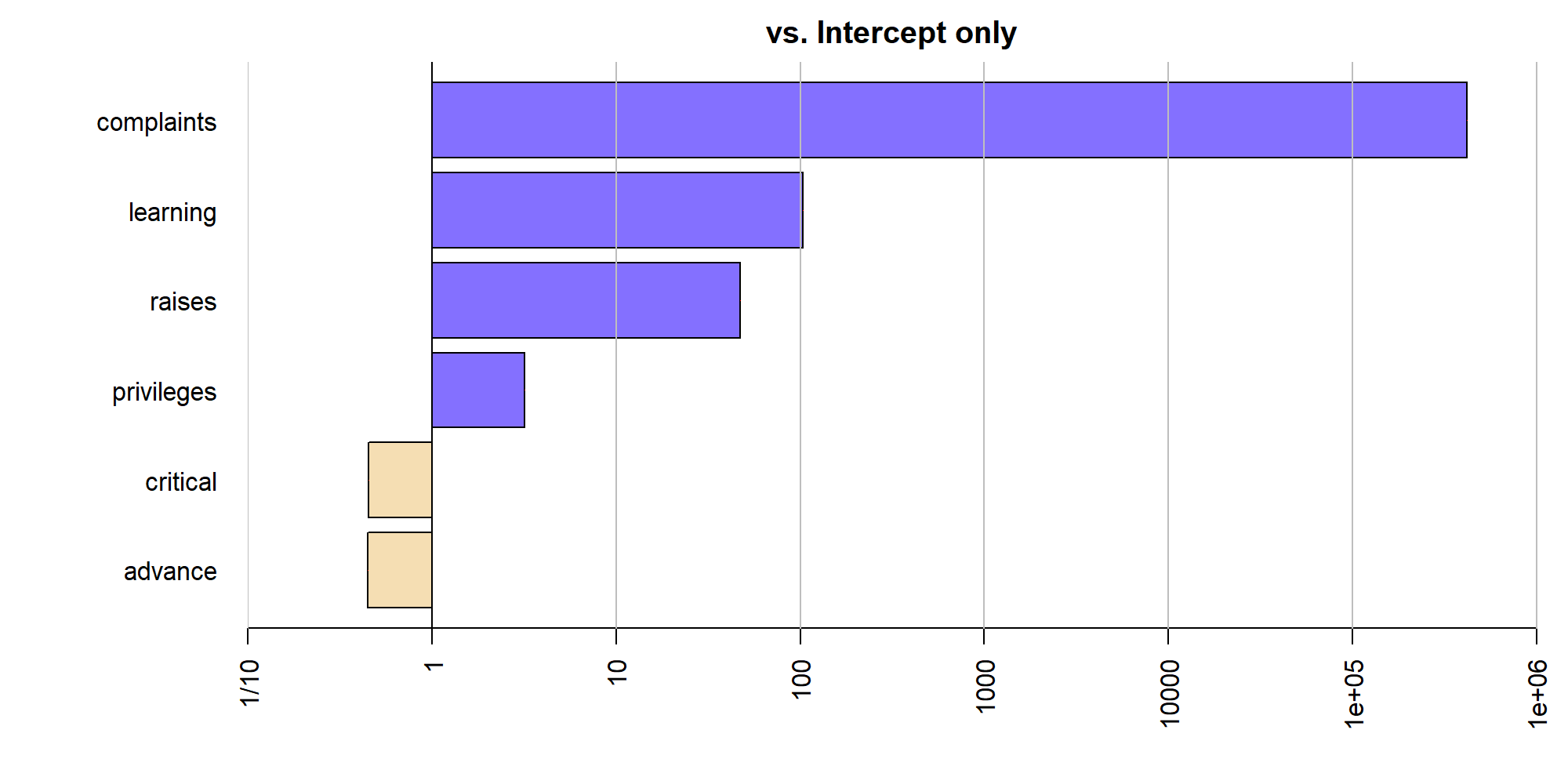The mismatch between the tests of all models, the “top-down” test, and the “bottom-up” test shows that the covariates share variance with one another. As always, whether these tests are interpretable or useful will depend on the data at hand.

In cases where it is desired to only compare a small number of models, the lmBF function can be used. Consider the case that we wish to compare the model containing only complaints to the model containing complaints and learning:

complaintsOnlyBf = lmBF(rating ~ complaints, data = attitude)
complaintsLearningBf = lmBF(rating ~ complaints + learning, data = attitude)
## Compare the two models
complaintsOnlyBf / complaintsLearningBf

## Bayes factor analysis
## --------------
##  complaints : 2.02 ±0.01%
##
## Against denominator:
##   rating ~ complaints + learning
## ---
## Bayes factor type: BFlinearModel, JZS


The complaints-only model is slightly preferred.

As with the other Bayes factors, it is possible to sample from the posterior distribution of a particular model under consideration. If we wanted to sample from the posterior distribution of the complaints + learning model, we could use the posterior function:

chains = posterior(complaintsLearningBf, iterations = 10000)
summary(chains)

##
## Iterations = 1:10000
## Thinning interval = 1
## Number of chains = 1
## Sample size per chain = 10000
##
## 1. Empirical mean and standard deviation for each variable,
##    plus standard error of the mean:
##
##              Mean     SD Naive SE Time-series SE
## mu         64.619  1.305  0.01305        0.01305
## complaints  0.609  0.127  0.00127        0.00133
## learning    0.201  0.139  0.00139        0.00139
## sig2       52.019 15.768  0.15768        0.19387
## g           1.972  7.349  0.07349        0.07611
##
## 2. Quantiles for each variable:
##
##               2.5%    25%    50%    75%  97.5%
## mu         62.0398 63.758 64.627 65.487 67.171
## complaints  0.3572  0.526  0.610  0.694  0.856
## learning   -0.0749  0.110  0.202  0.293  0.472
## sig2       29.6847 41.015 49.551 59.708 90.126
## g           0.1672  0.457  0.840  1.679  9.252


Compare these to the corresponding results from the classical regression analysis:

summary(lm(rating ~ complaints + learning, data = attitude))

##
## Call:
## lm(formula = rating ~ complaints + learning, data = attitude)
##
## Residuals:
##    Min     1Q Median     3Q    Max
## -11.56  -5.73   0.67   6.53  10.36
##
## Coefficients:
##             Estimate Std. Error t value Pr(>|t|)
## (Intercept)    9.871      7.061    1.40     0.17
## complaints     0.644      0.118    5.43  9.6e-06 ***
## learning       0.211      0.134    1.57     0.13
## ---
## Signif. codes:  0 '***' 0.001 '**' 0.01 '*' 0.05 '.' 0.1 ' ' 1
##
## Residual standard error: 6.82 on 27 degrees of freedom
## Multiple R-squared:  0.708,  Adjusted R-squared:  0.686
## F-statistic: 32.7 on 2 and 27 DF,  p-value: 6.06e-08


The results are quite similar, apart from the intercept. This is due to the Bayesian model centering the covariates before analysis, so the mu parameter is the mean of $$y$$ rather than the expected value of the response variable when all uncentered covariates are equal to 0.

## General linear models: mixing continuous and categorical covariates

The anovaBF and regressionBF functions are convenience functions designed to test several hypotheses of a particular type at once. Neither function allows the mixing of continuous and categorical covariates. If it is desired to test a model including both kinds of covariates, lmBF function must be used. We will continue the ToothGrowth example, this time without converting dose to a categorical variable. Instead, we will model the logarithm of the dose.

data(ToothGrowth)

# model log2 of dose instead of dose directly
ToothGrowth$dose = log2(ToothGrowth$dose)

# Classical analysis for comparison
lmToothGrowth <- lm(len ~ supp + dose + supp:dose, data=ToothGrowth)
summary(lmToothGrowth)

##
## Call:
## lm(formula = len ~ supp + dose + supp:dose, data = ToothGrowth)
##
## Residuals:
##    Min     1Q Median     3Q    Max
## -7.543 -2.492 -0.503  2.712  7.857
##
## Coefficients:
##             Estimate Std. Error t value Pr(>|t|)
## (Intercept)   20.663      0.679   30.43  < 2e-16 ***
## suppVC        -3.700      0.960   -3.85   0.0003 ***
## dose           6.415      0.832    7.71  2.3e-10 ***
## suppVC:dose    2.665      1.176    2.27   0.0274 *
## ---
## Signif. codes:  0 '***' 0.001 '**' 0.01 '*' 0.05 '.' 0.1 ' ' 1
##
## Residual standard error: 3.72 on 56 degrees of freedom
## Multiple R-squared:  0.776,  Adjusted R-squared:  0.764
## F-statistic: 64.5 on 3 and 56 DF,  p-value: <2e-16


The classical analysis, presented for comparison, reveals extremely low p values for the effects of the supplement type and of the dose, but the interaction p value is more moderate, at about 0.03. We can use the lmBF function to compute the Bayes factors for all models of interest against the null model, which in this case is the intercept-only model. We then concatenate them into a single Bayes factor object for convenience.

full <- lmBF(len ~ supp + dose + supp:dose, data=ToothGrowth)
noInteraction <- lmBF(len ~ supp + dose, data=ToothGrowth)
onlyDose <- lmBF(len ~ dose, data=ToothGrowth)
onlySupp <- lmBF(len ~ supp, data=ToothGrowth)

allBFs <- c(full, noInteraction, onlyDose, onlySupp)
allBFs

## Bayes factor analysis
## --------------
##  supp + dose + supp:dose : 1.58e+15 ±1.08%
##  supp + dose             : 1.49e+15 ±1.33%
##  dose                    : 2.77e+13 ±0.01%
##  supp                    : 1.2      ±0.01%
##
## Against denominator:
##   Intercept only
## ---
## Bayes factor type: BFlinearModel, JZS


The highest two Bayes factors belong to the full model and the model with no interaction. We can directly compute the Bayes factor for the simpler model with no interaction against the full model:

full / noInteraction

## Bayes factor analysis
## --------------
##  supp + dose + supp:dose : 1.06 ±1.72%
##
## Against denominator:
##   len ~ supp + dose
## ---
## Bayes factor type: BFlinearModel, JZS


The evidence here is clearly equivocal. We can also use the posterior function to compute parameter estimates.

chainsFull <- posterior(full, iterations = 10000)

# summary of the "interesting" parameters
summary(chainsFull[,1:7])

##
## Iterations = 1:10000
## Thinning interval = 1
## Number of chains = 1
## Sample size per chain = 10000
##
## 1. Empirical mean and standard deviation for each variable,
##    plus standard error of the mean:
##
##                      Mean    SD Naive SE Time-series SE
## mu                  18.81 0.500  0.00500        0.00500
## supp-OJ              1.68 0.501  0.00501        0.00546
## supp-VC             -1.68 0.501  0.00501        0.00546
## dose-dose            7.62 0.609  0.00609        0.00627
## supp:dose-OJ.&.dose -1.32 0.596  0.00596        0.00596
## supp:dose-VC.&.dose  1.32 0.596  0.00596        0.00596
## sig2                14.70 2.900  0.02900        0.03299
##
## 2. Quantiles for each variable:
##
##                       2.5%    25%   50%    75%  97.5%
## mu                  17.830 18.481 18.82 19.145 19.784
## supp-OJ              0.711  1.345  1.68  2.019  2.664
## supp-VC             -2.664 -2.019 -1.68 -1.345 -0.711
## dose-dose            6.428  7.209  7.61  8.029  8.816
## supp:dose-OJ.&.dose -2.503 -1.713 -1.32 -0.923 -0.117
## supp:dose-VC.&.dose  0.117  0.923  1.32  1.713  2.503
## sig2                10.113 12.627 14.35 16.376 21.180


The left panel of the figure below shows the data and linear fits. The green points represent guinea pigs given the orange juice supplement (OJ); red points represent guinea pigs given the vitamin C supplement. The solid lines show the posterior means from the Bayesian model; the dashed lines show the classical least-squares fit when applied to each supplement separately. The fits are quite close.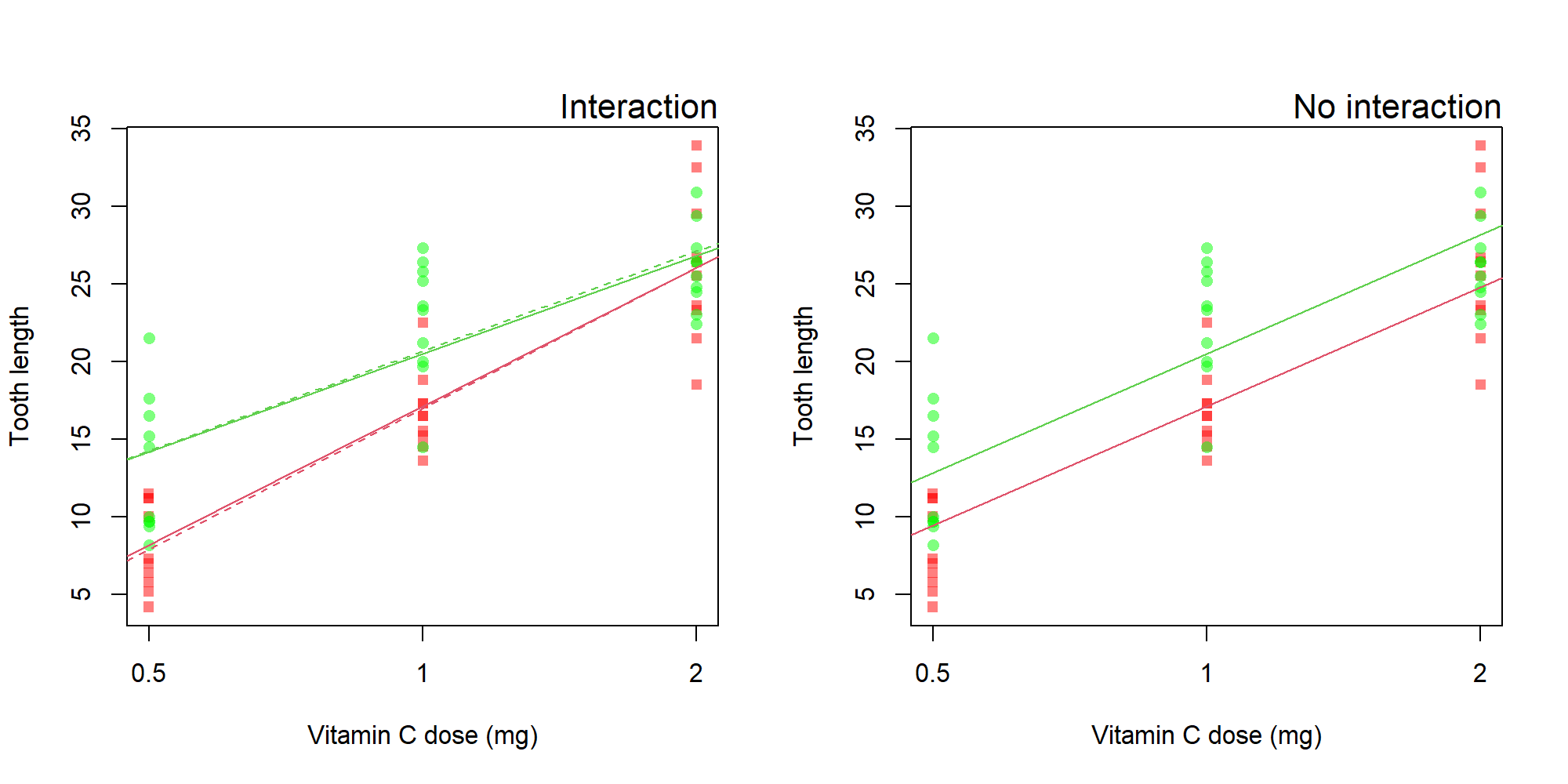Because the no-interaction model fares so well against the interaction model, it may be instructive to examine the fit of the no-interaction model. We sample from the no-interaction model with the posterior function:

chainsNoInt <- posterior(noInteraction, iterations = 10000)

# summary of the "interesting" parameters
summary(chainsNoInt[,1:5])

##
## Iterations = 1:10000
## Thinning interval = 1
## Number of chains = 1
## Sample size per chain = 10000
##
## 1. Empirical mean and standard deviation for each variable,
##    plus standard error of the mean:
##
##            Mean    SD Naive SE Time-series SE
## mu        18.81 0.508  0.00508        0.00508
## supp-OJ    1.67 0.511  0.00511        0.00568
## supp-VC   -1.67 0.511  0.00511        0.00568
## dose-dose  7.66 0.631  0.00631        0.00631
## sig2      15.67 3.060  0.03060        0.03395
##
## 2. Quantiles for each variable:
##
##             2.5%   25%   50%   75%  97.5%
## mu        17.830 18.47 18.81 19.15 19.812
## supp-OJ    0.692  1.32  1.67  2.01  2.692
## supp-VC   -2.692 -2.01 -1.67 -1.32 -0.692
## dose-dose  6.445  7.23  7.65  8.07  8.921
## sig2      10.832 13.50 15.26 17.41 22.690


The right panel of the figure above shows the fit of the no-interaction model to the data. This model appears to account for the data satisfactorily. Though the moderate p value of the classical result might lead us to reject the no-interaction model, the Bayes factor and the visual fit appear to agree that the evidence is equivocal at best.

We have now analyzed the ToothGrowth data using both ANOVA (with dose as a factor) and regression (with dose as a continuous covariate). We may wish to compare the two approaches. We first create a column of the data with dose as a factor, then use anovaBF:

ToothGrowth$doseAsFactor <- factor(ToothGrowth$dose)
levels(ToothGrowth$doseAsFactor) <- c(.5,1,2) aovBFs <- anovaBF(len ~ doseAsFactor + supp + doseAsFactor:supp, data = ToothGrowth)  Because all models we've considered are compared to the null intercept-only model, we can concatenate the aovBFs object with the Bayes factors we previously computed in this section: allBFs <- c(aovBFs, full, noInteraction, onlyDose) ## eliminate the supp-only model, since it performs so badly allBFs <- allBFs[-1] ## Compare to best model allBFs / max(allBFs)  ## Bayes factor analysis ## -------------- ##  doseAsFactor : 0.00315 ±1.08% ##  supp + doseAsFactor : 0.182 ±2.51% ##  supp + doseAsFactor + supp:doseAsFactor : 0.488 ±2.02% ##  supp + dose + supp:dose : 1 ±0% ##  supp + dose : 0.939 ±1.72% ##  dose : 0.0175 ±1.08% ## ## Against denominator: ## len ~ supp + dose + supp:dose ## --- ## Bayes factor type: BFlinearModel, JZS  Two of the models score essentially equally well in terms of Bayes factors: supp + dose + supp:dose and supp + dose, suggesting that the interaction adds little. The Bayes factors where dose is treated as a factor are all worse than when dose is treated as a continuous covariate. This is likely due to a the added flexibility allowed by including more parameters. Plotting the Bayes factors shows how large the differences are: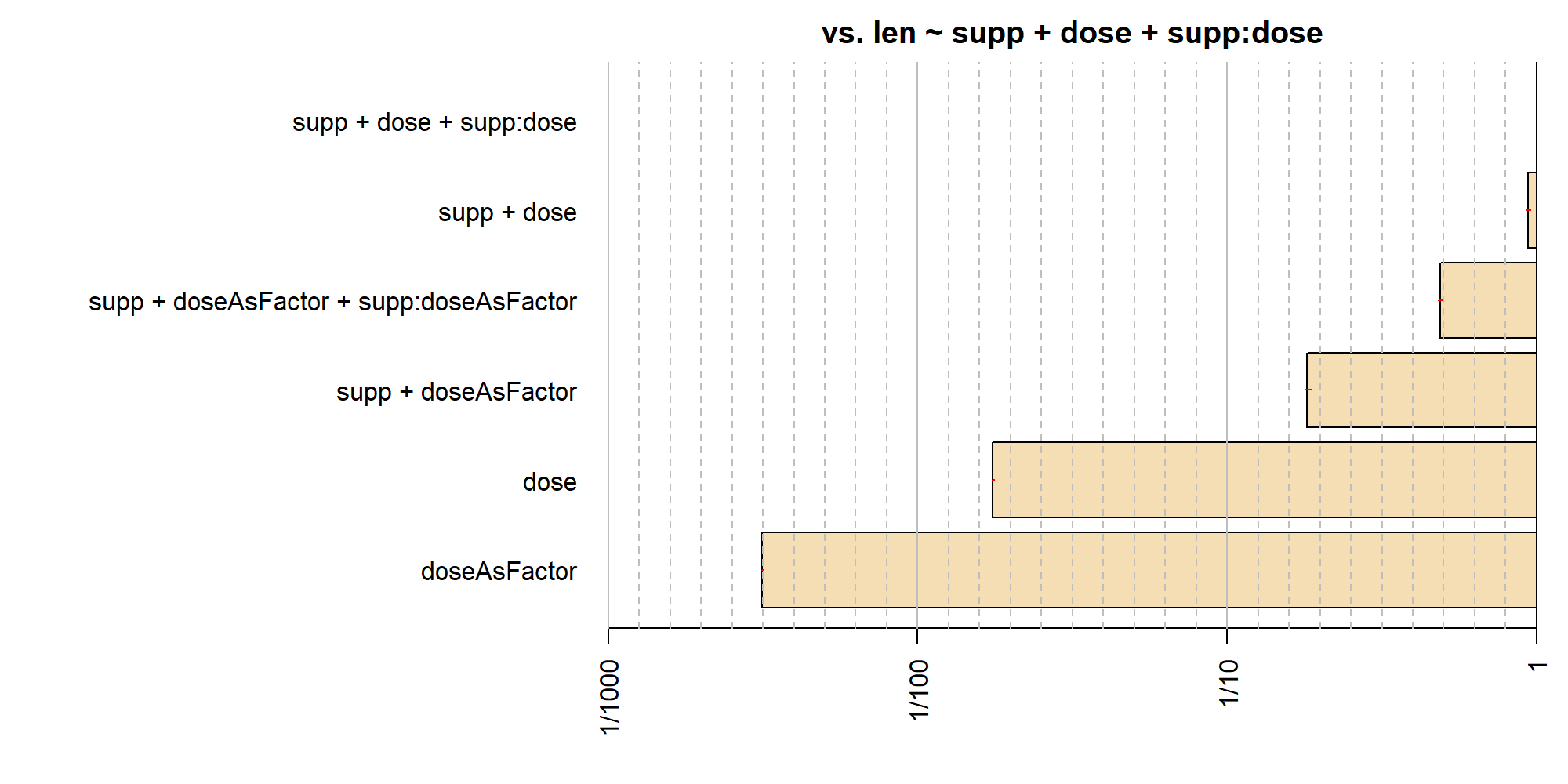#### Linear correlation (0.9.12-4+) Ly, Verhagen, and Wagenmakers (2015; link) present a Bayes factor test for linear correlation. The BayesFactor package allows the computing of the Bayes factor and sampling from the posterior of the Bayes factor. Note that the model and priors are somewhat different from those used in the linear regression models presented above; further discussion can be found in Ly et al. We demonstrate the use of the correlationBF function using Fisher's iris data set built into R. See the help (?iris in R) for more details. We will focus on the correlation between Sepal.Length and Sepal.Width. First, we create a scatterplot. plot(Sepal.Width ~ Sepal.Length, data = iris) abline(lm(Sepal.Width ~ Sepal.Length, data = iris), col = "red")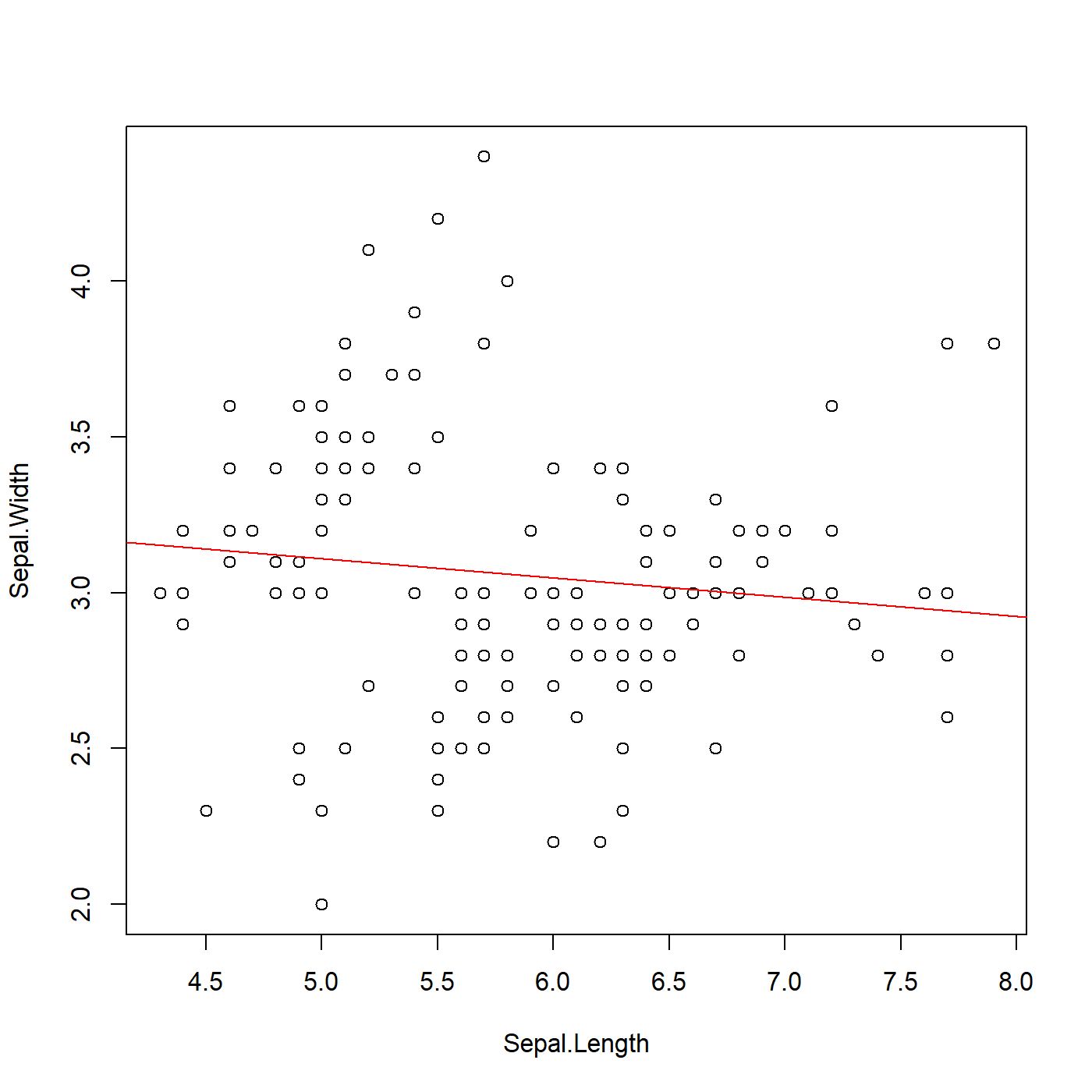There does not appear to be a substantial correlation between these two variables. We can compute a classical test of the correlation using R's cor.test function: cor.test(y = iris$Sepal.Length, x = iris$Sepal.Width)  ## ## Pearson's product-moment correlation ## ## data: iris$Sepal.Width and iris$Sepal.Length ## t = -1, df = 148, p-value = 0.2 ## alternative hypothesis: true correlation is not equal to 0 ## 95 percent confidence interval: ## -0.2727 0.0435 ## sample estimates: ## cor ## -0.118  The $$p$$ value is nonsignificant at typical $$\alpha$$ levels, and the point estimate is not terribly impressive at -0.12. To compute the corresponding Bayes factor test, we use the correlationBF function (note the default prior scale). bf = correlationBF(y = iris$Sepal.Length, x = iris$Sepal.Width) bf  ## Bayes factor analysis ## -------------- ##  Alt., r=0.333 : 0.509 ±0% ## ## Against denominator: ## Null, rho = 0 ## --- ## Bayes factor type: BFcorrelation, Jeffreys-beta*  As would be expected from the middling $$p$$ value in the classical test, the Bayes factor test shows little evidence either way (about 2 in favor of the null). If we'd like to estimate the correlation on the assumption that it is non-zero, we can sample from the posterior distribution using the posterior function. samples = posterior(bf, iterations = 10000)  ## Independent-candidate M-H acceptance rate: 96%  The important parameter is rho, the estimate of the true linear correlation. summary(samples)  ## ## Iterations = 1:10000 ## Thinning interval = 1 ## Number of chains = 1 ## Sample size per chain = 10000 ## ## 1. Empirical mean and standard deviation for each variable, ## plus standard error of the mean: ## ## Mean SD Naive SE Time-series SE ## rho -0.111 0.0789 0.000789 0.000824 ## zeta -0.112 0.0803 0.000803 0.000839 ## ## 2. Quantiles for each variable: ## ## 2.5% 25% 50% 75% 97.5% ## rho -0.260 -0.165 -0.111 -0.0576 0.0454 ## zeta -0.266 -0.166 -0.112 -0.0577 0.0454  The posterior mean and credible interval for rho are very close to the point estimate and confidence interval obtained from cor.test. We can also plot the full posterior distribution, if we like: plot(samples[,"rho"])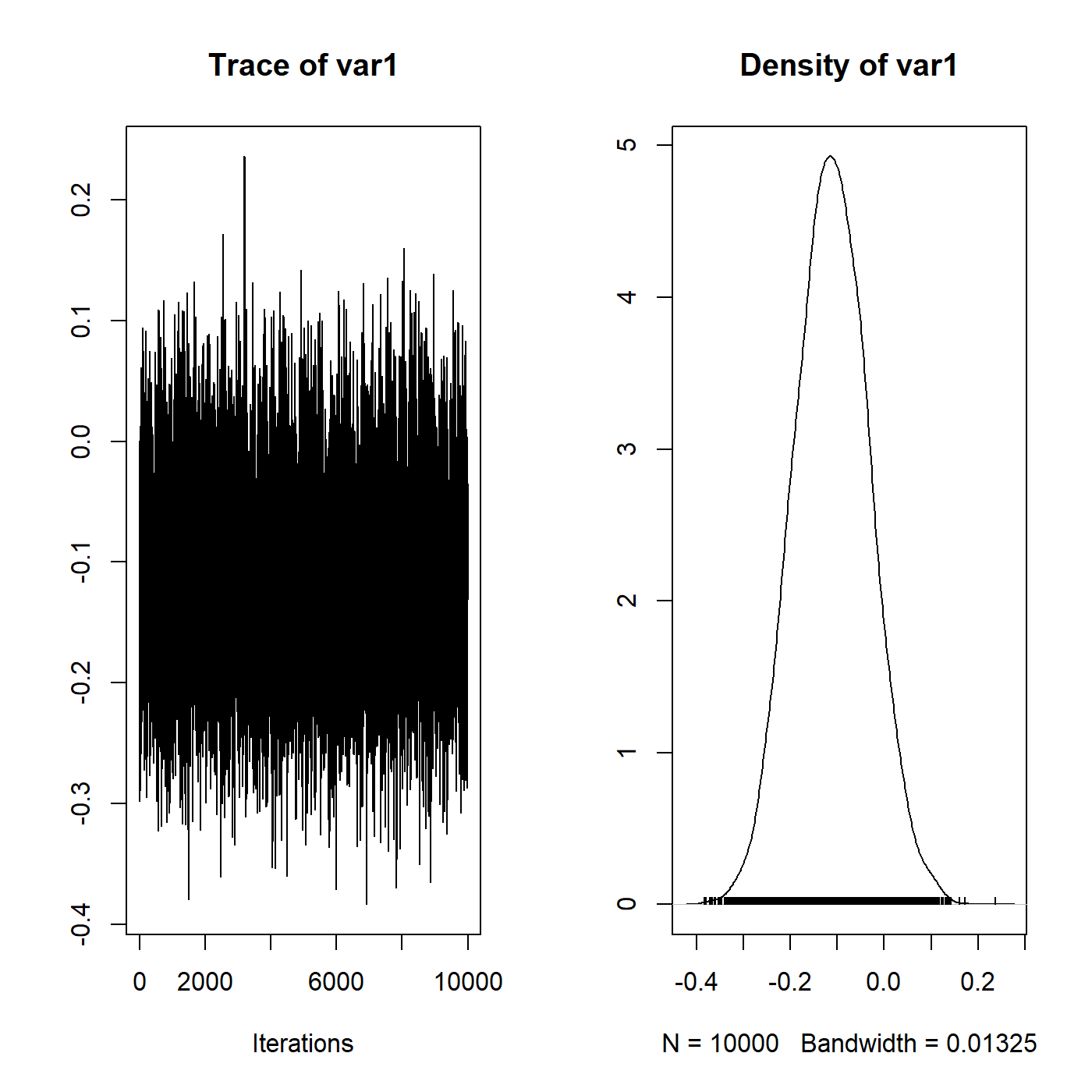### Tests of single proportions (0.9.9+) The default test for a proportion assumes that all observations were independent with fixed probability $$\pi$$. The rule for stopping can be fixed $$N$$ (binomial sampling) or a fixed number of successes (negative binomial sampling); unlike a significance test, the Bayes factor does not depend on the stopping rule. For the Bayes factor test of a single proportion, there are two hypotheses; the null hypothesis assumes that the probability $$\pi$$ is a fixed, known value $$p$$; under the alternative, the log-odds corresponding to $$\pi$$, denoted $$\omega = \log(\pi/(1-\pi))$$, has a logistic distribution centered on the log-odds corresponding to the null value $$p$$ (denoted $$\omega_0 = \log(p/(1-p))$$: $\omega \sim \mbox{logistic}(\mbox{mean}=\omega_0, \mbox{scale}=r)$ The default prior $$r$$ scale is ½. The figure below shows the prior distribution assuming the null hypothesis $$p=0.5$$, for the three named prior scale settings $$r$$ (“medium”, “wide”, and “ultrawide”). The default is “medium”: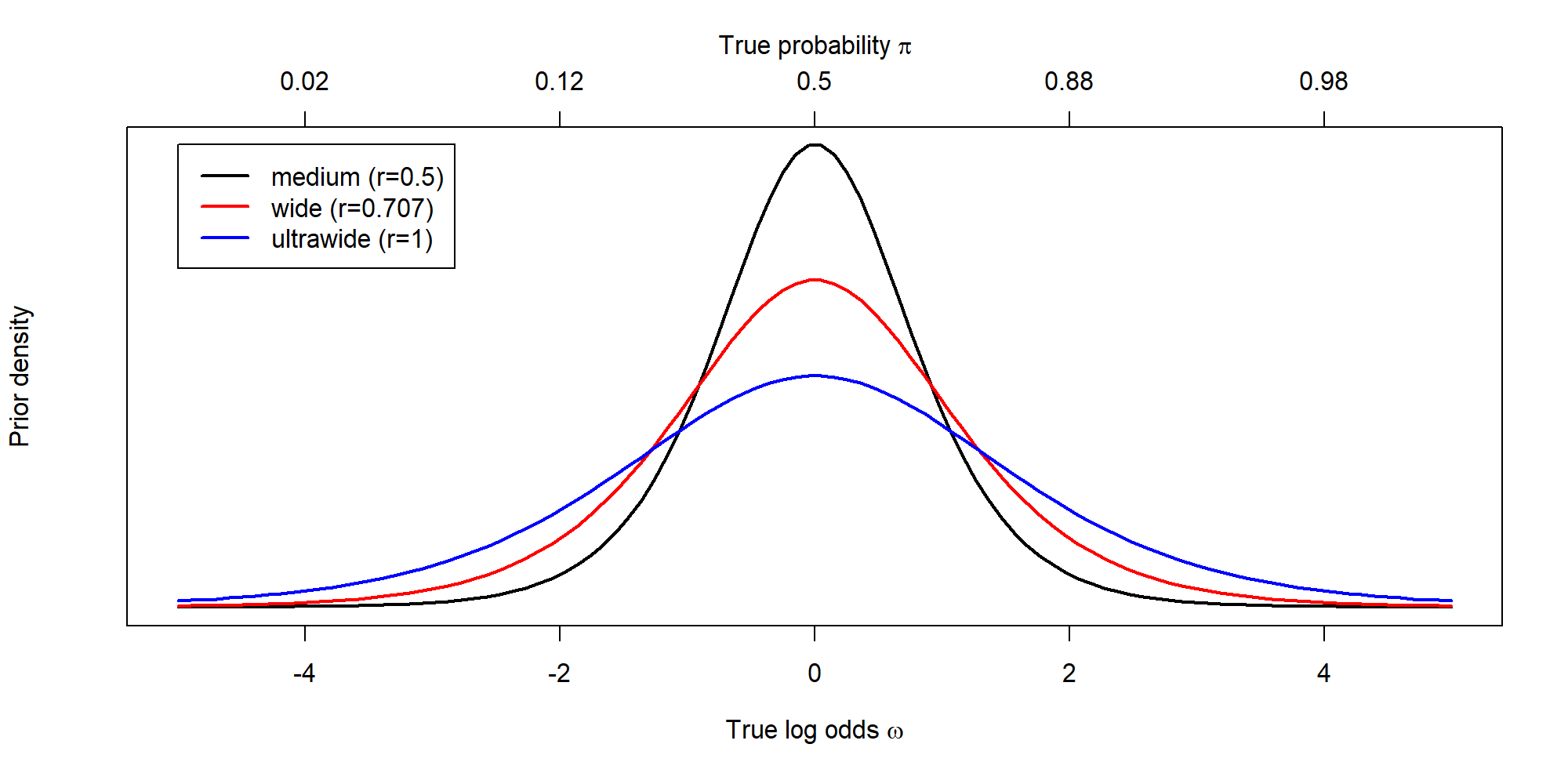The following example is taken from ?binom.test, which cites Conover (1971). Under (the assumption of) simple Mendelian inheritance, a cross between plants of two particular genotypes produces progeny ¼ of which are “dwarf” and ¾ of which are “giant”, respectively. In an experiment to determine if this assumption is reasonable, a cross results in progeny having 243 dwarf and 682 giant plants. If “giant” is taken as success, the null hypothesis is that $$p = ¾$$ and the alternative that $$p \neq ¾$$. bf = proportionBF( 682, 682 + 243, p = 3/4) 1 / bf  ## Bayes factor analysis ## -------------- ##  Null, p=0.75 : 7.27 ±0.01% ## ## Against denominator: ## Alternative, p0 = 0.75, r = 0.5, p =/= p0 ## --- ## Bayes factor type: BFproportion, logistic  The Bayes factor favors the null hypothesis by a factor of about 7 (which is not surprising given that the observed proportion is 73.7%). In contrast, the best we can say about the classical result is that it is not statistically “significant”: binom.test(682, 682 + 243, p = 3/4)  ## ## Exact binomial test ## ## data: 682 and 682 + 243 ## number of successes = 682, number of trials = 925, p-value = 0.4 ## alternative hypothesis: true probability of success is not equal to 0.75 ## 95 percent confidence interval: ## 0.708 0.765 ## sample estimates: ## probability of success ## 0.737  Using the posterior function, we can draw samples from the posterior distribution of the true log odds and true probability and plot the estimate of the posterior. chains = posterior(bf, iterations = 10000)  ## Independent-candidate M-H acceptance rate: 97%  plot(chains[,"p"], main = "Posterior of true probability\nof 'giant' progeny")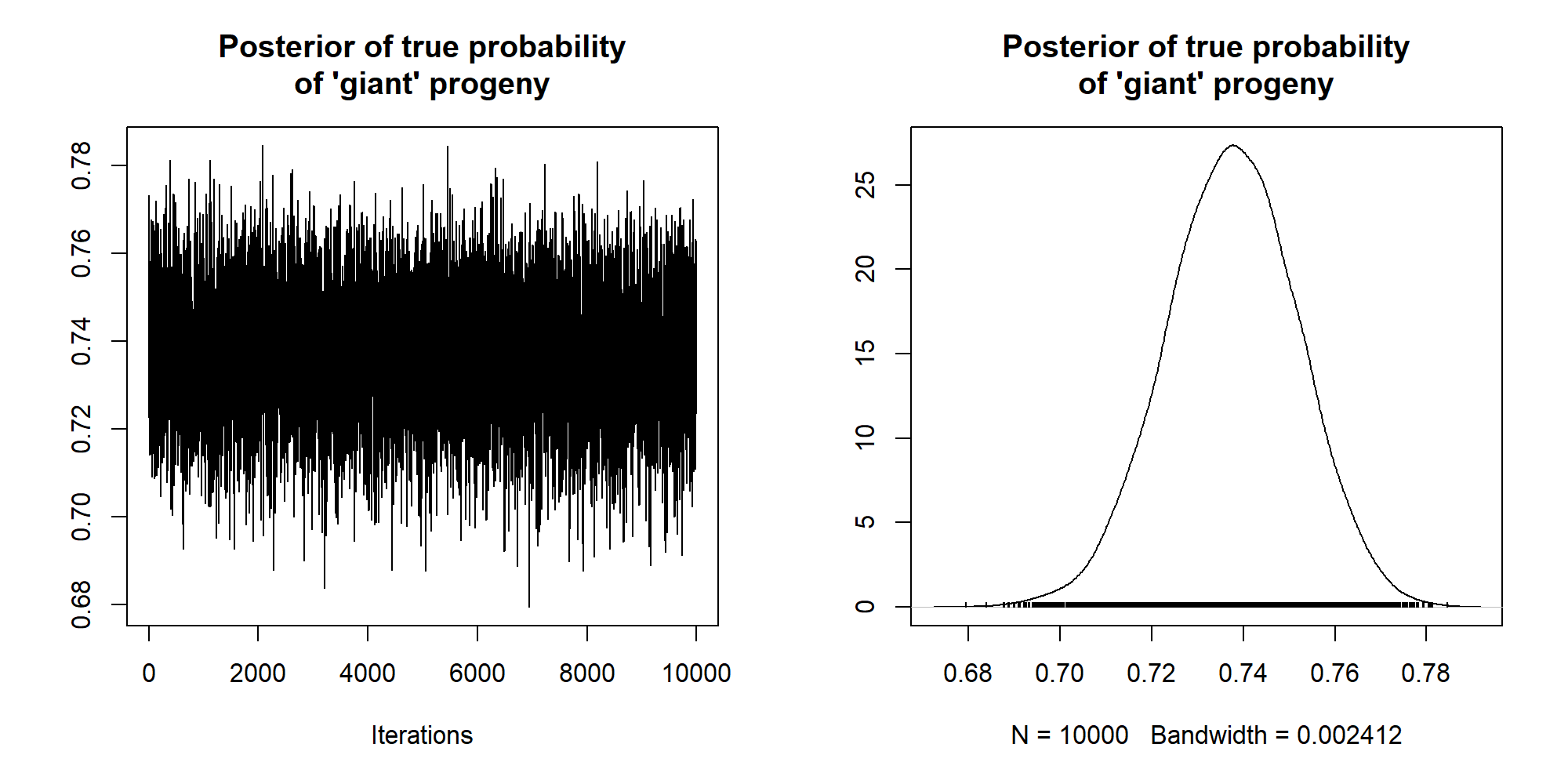### Contingency tables (0.9.9+) The BayesFactor package implements versions of Gunel and Dickey's (1974) contingency table Bayes factor tests. Bayes factors for contingency tests are computed using the contingencyTableBF function. The necessary arguments are a matrix of cell frequencies and details about the sampling plan that produced the data. Here, we provide an example analysis of Hraba and Grant's (1970) data, included as part of the BayesFactor package as the raceDolls data set. 71 white children and 89 black children from Lincoln, Nebraska were offered two dolls, one of whose “race” was the same as the child's and one that was different (either white or black). The children were then asked to select one of the dolls, with prompts such as “Give me the doll that is a nice doll.” 50 of the 71 white children (70%) selected the white doll, while 48 of the 89 black children (54%) selected the black doll. These data are shown in the table below: White child Black child Same-race doll 50 48 Different-race doll 21 41 We can perform a Bayes factor analysis using the contingencyTableBF function: bf = contingencyTableBF(raceDolls, sampleType = "indepMulti", fixedMargin = "cols") bf  ## Bayes factor analysis ## -------------- ##  Non-indep. (a=1) : 1.81 ±0% ## ## Against denominator: ## Null, independence, a = 1 ## --- ## Bayes factor type: BFcontingencyTable, independent multinomial  Here we used sampleType="indepMulti" and fixedMargin="cols" to specify that the columns are assumed to be sampled as independent multinomials with their total fixed. See the help at ?contingencyTableBF for more details about possible sampling plans and the priors. The Bayes factor in favor of the alternative that the factors are not independent is just shy of 2, which is not very much evidence against the null hypothesis. For comparison, consider the results classical chi-square test, with continuity correction: chisq.test(raceDolls)  ## ## Pearson's Chi-squared test with Yates' continuity correction ## ## data: raceDolls ## X-squared = 4, df = 1, p-value = 0.05  The classical test is just barely statistically significant. We can also use the posterior function to estimate the difference in probabilities of selecing a doll of the same race between white and black children, assuming the non-independence alternative: chains = posterior(bf, iterations = 10000)  For the independent multinomial sampling plan, the chains will contain the individual cell probabilities and the marginal column probabilities. We first need to compute the conditional probabilities from the results: sameRaceGivenWhite = chains[,"pi[1,1]"] / chains[,"pi[*,1]"] sameRaceGivenBlack = chains[,"pi[1,2]"] / chains[,"pi[*,2]"]  …and then plot the MCMC estimate of the difference: plot(mcmc(sameRaceGivenWhite - sameRaceGivenBlack), main = "Increase in probability of child picking\nsame race doll (white - black)")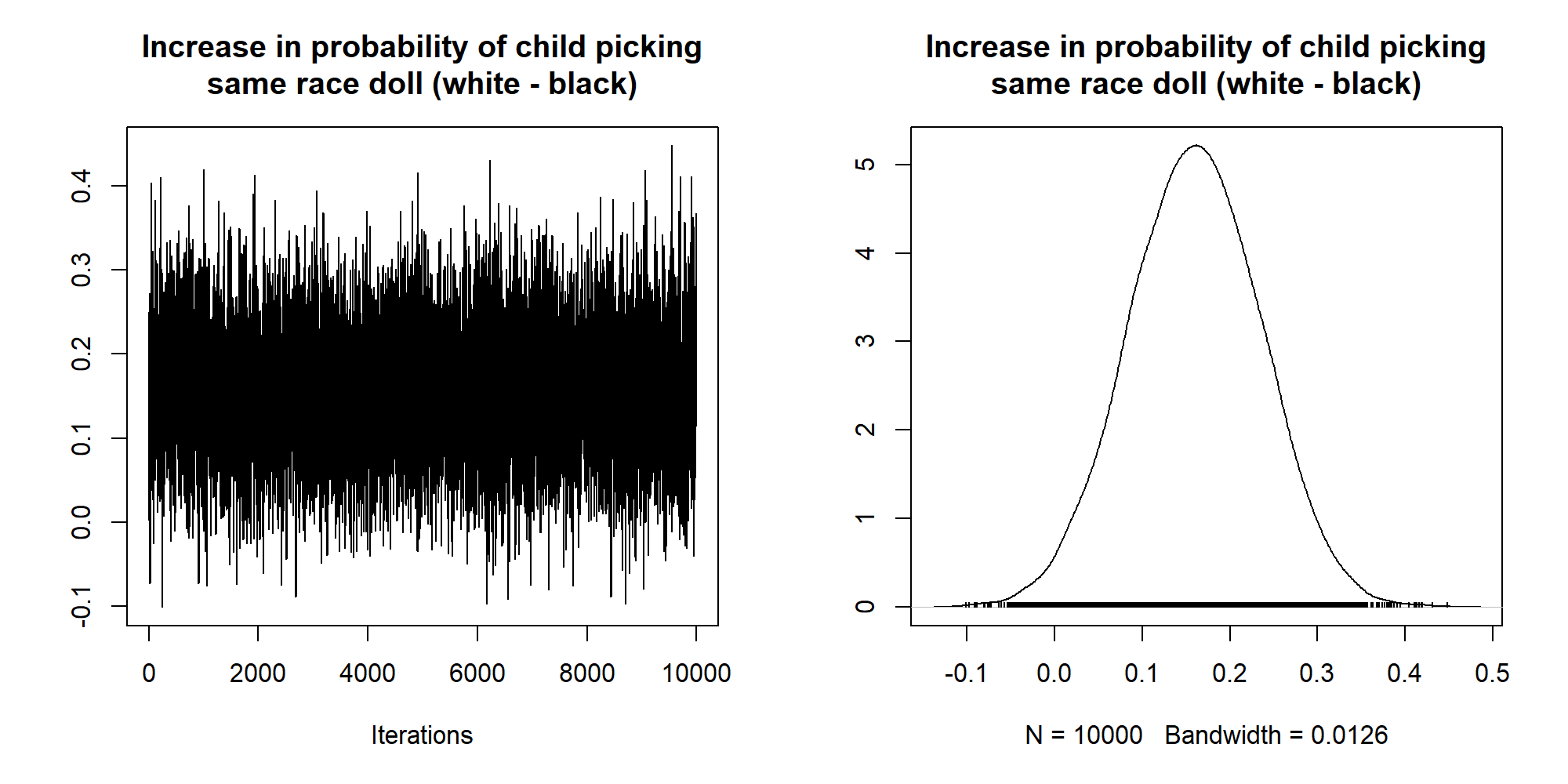For more information, see ?contingencyTableBF. ## Additional tips and tricks (0.9.4+) In this section, tricks to help save time and memory are described. These tricks work with version BayesFactor version 0.9.4+, unless otherwise indicated. ### Testing restrictions on linear models: generalTestBF The convienience functions anovaBF and regressionBF are specifically designed for cetagorical and continuous covariates respectively, and have limitations that make those functions easier to use. For instance, anovaBF cannot incorporate continuous covariates, and treats random effects as untested nuissance parameters. The regressionBF on the other hand, being strictly for multiple regression, cannot incorporate categorical covariates. These functions exist for particular purposes, since guessing what model comparisons a user wants in general is difficult. The lmBF function, on the other hand, can handle any model but is limited to a single model comparison: the specified model against the intercept-only model. The generalTestBF function allows the testing of groups of models (like anovaBF and regressionBF) but can handle any kind of model (like lmBF). Users specify a full model, and generalTestBF successively removes terms from that model and tests the resulting submodels. For example, using the puzzles data set described above: data(puzzles) puzzleGenBF <- generalTestBF(RT ~ shape + color + shape:color + ID, data=puzzles, whichRandom="ID") puzzleGenBF  ## Bayes factor analysis ## -------------- ##  shape : 0.611 ±0.01% ##  color : 0.611 ±0.01% ##  shape + color : 0.382 ±0.83% ##  shape + color + shape:color : 0.134 ±2.18% ##  ID : 111517 ±0% ##  shape + ID : 318608 ±1.12% ##  color + ID : 312963 ±0.93% ##  shape + color + ID : 1320210 ±2.27% ##  shape + color + shape:color + ID : 509046 ±7.21% ## ## Against denominator: ## Intercept only ## --- ## Bayes factor type: BFlinearModel, JZS  The resulting 9 models are the full model, plus the models that can be built by removing a single term at a time from the full model. By default, the generalTestBF function will not eliminate a term that is involved in a higher-order interaction (for instance, we will not remove shape unless the shape:color interaction is also removed); this behavior can be modified through the whichModels argument. It is often the case that some terms are nuisance terms that we would like to always keep in the model. For instance, ID in the puzzles data set is a participant effect; we would not generally consider models without a participant effect to be plausible. We can use the neverExclude argument to the function to specify a set of search terms (technically, extended regular expressions) that, if matched, will specify that the term is always to be kept, and never excluded. To keep the ID term: puzzleGenBF <- generalTestBF(RT ~ shape + color + shape:color + ID, data=puzzles, whichRandom="ID", neverExclude="ID") puzzleGenBF  ## Bayes factor analysis ## -------------- ##  shape + ID : 318624 ±1.25% ##  color + ID : 315164 ±1.09% ##  shape + color + ID : 1287049 ±2.7% ##  shape + color + shape:color + ID : 465008 ±1.99% ##  ID : 111517 ±0% ## ## Against denominator: ## Intercept only ## --- ## Bayes factor type: BFlinearModel, JZS  The function now only considers models that contain ID. In some cases — especially when variable names are short, or a term to be kept is part of an interaction term that can be eliminated — we need to be careful in specifying search terms using neverExclude. For instance, suppose we are interested in testing the ID:shape interaction puzzleGenBF <- generalTestBF(RT ~ shape + color + shape:color + shape:ID + ID, data=puzzles, whichRandom="ID", neverExclude="ID") puzzleGenBF  ## Bayes factor analysis ## -------------- ##  color + shape + ID + shape:ID : 174461 ±2.88% ##  color + color:shape + shape + ID + shape:ID : 63932 ±2.28% ##  shape + ID + shape:ID : 28761 ±1.09% ## ## Against denominator: ## Intercept only ## --- ## Bayes factor type: BFlinearModel, JZS  The shape:ID interaction is never eliminated, because it matches the ID search term from neverExclude. Regular expressions are useful here. There are special characters representing the beginning and ending of a string (^ and $, respectively) that we can use to construct a regular expression that will match ID but not shape:ID:

puzzleGenBF <- generalTestBF(RT ~ shape + color + shape:color + shape:ID + ID, data=puzzles, whichRandom="ID", neverExclude="^ID$") puzzleGenBF  ## Bayes factor analysis ## -------------- ##  shape + ID : 310892 ±0.73% ##  color + ID : 313151 ±0.98% ##  shape + color + ID : 1610764 ±19.8% ##  shape + color + shape:color + ID : 454583 ±1.56% ##  shape + shape:ID + ID : 28852 ±0.95% ##  shape + color + shape:ID + ID : 169313 ±1.25% ##  shape + color + shape:color + shape:ID + ID : 63199 ±2.38% ##  ID : 111517 ±0% ## ## Against denominator: ## Intercept only ## --- ## Bayes factor type: BFlinearModel, JZS  The shape:ID interaction term is now eliminated in some models, because it does not match "^ID$". Multiple terms may be provided to neverExclude by providing a character vector; terms which match any element in the vector will always be included in model comparisons.

### Saving time: Pre-culling Bayes factor objects

In cases where the default analysis produces many models to compare, the sampling approach to computing Bayes factors can be time consuming. The BayesFactor package identifies situations where sampling is not needed and thus saves time, but any model in which there is more than one categorical factor or a mix of categorical and continuous predictors will require sampling. When a default analysis produces many models that are not of interest, much of the time spent sampling may be wasted.

The main functions in the BayesFactor package include the noSample argument which, if true, will prevent sampling. If a Bayes factor can be computed without sampling, the package will compute it, returning NA for Bayes factors that would require sampling. Continuing using the puzzles dataset:

puzzleCullBF <- generalTestBF(RT ~ shape + color + shape:color + ID, data=puzzles, whichRandom="ID", noSample=TRUE,whichModels='all')

puzzleCullBF

## Bayes factor analysis
## --------------
##  shape                             : 0.611  ±0.01%
##  color                             : 0.611  ±0.01%
##  ID                                : 111517 ±0%
##  shape:color                       : 0.287  ±0.01%
##  shape + color                     : NA     ±NA%
##  shape + ID                        : NA     ±NA%
##  shape + shape:color               : NA     ±NA%
##  color + ID                        : NA     ±NA%
##  color + shape:color               : NA     ±NA%
##  ID + shape:color                 : NA     ±NA%
##  shape + color + ID               : NA     ±NA%
##  shape + color + shape:color      : NA     ±NA%
##  shape + ID + shape:color         : NA     ±NA%
##  color + ID + shape:color         : NA     ±NA%
##  shape + color + ID + shape:color : NA     ±NA%
##
## Against denominator:
##   Intercept only
## ---
## Bayes factor type: BFlinearModel, JZS


Here we use whichModels='all' for demonstration, in order to obtain more possible model comparisons. Notice that several of the Bayes factors were computable without sampling, and are reported. The others have missing values, because the Bayes factor would have required sampling to compute.

For now, we can separate the missing and non-missing Bayes factors in separate variables. This is made easy by the is.na method for BayesFactor objects:

missing = puzzleCullBF[ is.na(puzzleCullBF) ]
done = puzzleCullBF[ !is.na(puzzleCullBF) ]

missing

## Bayes factor analysis
## --------------
##  shape + color                     : NA ±NA%
##  shape + ID                        : NA ±NA%
##  shape + shape:color               : NA ±NA%
##  color + ID                        : NA ±NA%
##  color + shape:color               : NA ±NA%
##  ID + shape:color                  : NA ±NA%
##  shape + color + ID                : NA ±NA%
##  shape + color + shape:color       : NA ±NA%
##  shape + ID + shape:color          : NA ±NA%
##  color + ID + shape:color         : NA ±NA%
##  shape + color + ID + shape:color : NA ±NA%
##
## Against denominator:
##   Intercept only
## ---
## Bayes factor type: BFlinearModel, JZS


The variable missing now contains all models for which we lack a Bayes factor. At this point, we decide which of the Bayes factors we would like to compute. We can do this in any way we like: we could simple specify a subset, like missing[1:3] or we could do something more complicated. Here, we will include based on the model formula, using the R function grepl (?grepl). Suppose we only wanted models that did not include both shape and color. First, we obtain the names of the models in missing, and then test the names to see if they match our restriction with grepl. We can use the result to restrict the models to compare to only those of interest.

# get the names of the numerator models
missingModels = names(missing)\$numerator

# search them to see if they contain "shape" or "color" -
# results are logical vectors
containsShape = grepl("shape",missingModels)
containsColor = grepl("color",missingModels)

# anything that does not contain "shape" and "color"
containsOnlyOne = !(containsShape & containsColor)

# restrict missing to only those of interest
missingOfInterest = missing[containsOnlyOne]

missingOfInterest

## Bayes factor analysis
## --------------
##  shape + ID : NA ±NA%
##  color + ID : NA ±NA%
##
## Against denominator:
##   Intercept only
## ---
## Bayes factor type: BFlinearModel, JZS


We have restricted our set down to 2 items from 11 items. We can now use recompute to compute the missing Bayes factors:

# recompute the Bayes factors for the missing models of interest
sampledBayesFactors = recompute(missingOfInterest)

sampledBayesFactors

## Bayes factor analysis
## --------------
##  shape + ID : 317471 ±0.95%
##  color + ID : 316296 ±2.53%
##
## Against denominator:
##   Intercept only
## ---
## Bayes factor type: BFlinearModel, JZS

# Add them together with our other Bayes factors, already computed:
completeBayesFactors = c(done, sampledBayesFactors)

completeBayesFactors

## Bayes factor analysis
## --------------
##  shape       : 0.611  ±0.01%
##  color       : 0.611  ±0.01%
##  ID          : 111517 ±0%
##  shape:color : 0.287  ±0.01%
##  shape + ID  : 317471 ±0.95%
##  color + ID  : 316296 ±2.53%
##
## Against denominator:
##   Intercept only
## ---
## Bayes factor type: BFlinearModel, JZS


Note that we're still left with one model that contains both shape and color, because it was computed without sampling. Assuming that we were not interested in any model containing both shape and color, however, we may have saved considerable time by not sampling to estimate their Bayes factors.

The noSample argument will also work with the sampling of posteriors. This is especially useful, for instance, if one would like to know what order the MCMC chain results will be output in before sampling.

### Saving memory: Thinning and filtering MCMC chains

Modern computer systems, which have many gigabytes of RAM, contain sufficient memory to perform analyses of moderate scale using the BayesFactor package. Some systems — particularly older 32-bit systems — are limited in the amount of memory that can address. Posterior sampling can create output that is hundreds of megabytes in size. If a user conducts several of these analyses, R may not have sufficient memory to store the results.

Consider, for instance, an analysis with 100 participants, 100 items, and two fixed effects with 3 levels each. We include all main effects in the model, as well as all two-way interactions (excluding the participant by item interaction). This results in 619 parameters. Because each number stored in an MCMC chain uses 8 bytes of memory, each iterations of the chain uses 8*619=4952 bytes. If a user then requests a 100,000 iteration MCMC chain — a large, but not unreasonably, sized MCMC chain — the resulting object will use about 500Mb of memory. This is most of the memory available to the default installation of R on a 32-bit Windows system. Even if a computer has a lot of memory, many of the parameters may not be interesting to the analyst. The participant and item effects, for instance, may be nuisance variation. If a user is not interested in the estimates, it is a waste of memory to include them in the MCMC chain.

The BayesFactor package includes several methods for reducing the size of MCMC chains: column filtering and chain thinning. Column filtering ensures that certain parameters do not appear in the output; thinning reduces the length of MCMC chains by only keeping some of the iterations.

#### Column filtering

Consider again the puzzles data set. We begin by sampling from the MCMC chain of the model with the main effect of shape and color, along with their interaction, plus a participant effect:

data(puzzles)

# Get MCMC chains corresponding to "full" model
# We prevent sampling so we can see the parameter names
# iterations argument is necessary, but not used
fullModel = lmBF(RT ~ shape + color + shape:color + ID, data = puzzles, noSample=TRUE, posterior = TRUE, iterations=3)

fullModel

## Object of class "mcmc"
## Markov Chain Monte Carlo (MCMC) output:
## Start = 1
## End = 2
## Thinning interval = 1
##      mu shape-round shape-square color-color color-monochromatic ID-1 ID-2 ID-3
## [1,] NA          NA           NA          NA                  NA   NA   NA   NA
## [2,] NA          NA           NA          NA                  NA   NA   NA   NA
##      ID-4 ID-5 ID-6 ID-7 ID-8 ID-9 ID-10 ID-11 ID-12 shape:color-round.&.color
## [1,]   NA   NA   NA   NA   NA   NA    NA    NA    NA                        NA
## [2,]   NA   NA   NA   NA   NA   NA    NA    NA    NA                        NA
##      shape:color-round.&.monochromatic shape:color-square.&.color
## [1,]                                NA                         NA
## [2,]                                NA                         NA
##      shape:color-square.&.monochromatic sig2 g_shape g_color g_ID g_shape:color
## [1,]                                 NA   NA      NA      NA   NA            NA
## [2,]                                 NA   NA      NA      NA   NA            NA
## ---
##  Model:
## Type: BFlinearModel, JZS
## RT ~ shape + color + shape:color + ID
## Data types:
## ID :  fixed
## shape :  fixed
## color :  fixed


Notice that the participant effects, which are often regarded as nuisance, are included in the chain. These parameters double the size of the MCMC object; if we are not interested in the parameter values, we could eliminate them from the output for a considerable savings. This does not mean, however, that the parameters are not estimated; they will still be used by BayesFactor, but will not be reported.

To do this, we pass the columnFilter argument to the sampler, which surpresses output of any columns that arise from a term matched by an element in columnFilter.

fullModelFiltered = lmBF(RT ~ shape + color + shape:color + ID, data = puzzles, noSample=TRUE, posterior = TRUE, iterations=3,columnFilter="ID")

fullModelFiltered

## Object of class "mcmc"
## Markov Chain Monte Carlo (MCMC) output:
## Start = 1
## End = 2
## Thinning interval = 1
##      mu shape-round shape-square color-color color-monochromatic
## [1,] NA          NA           NA          NA                  NA
## [2,] NA          NA           NA          NA                  NA
##      shape:color-round.&.color shape:color-round.&.monochromatic
## [1,]                        NA                                NA
## [2,]                        NA                                NA
##      shape:color-square.&.color shape:color-square.&.monochromatic sig2 g_shape
## [1,]                         NA                                 NA   NA      NA
## [2,]                         NA                                 NA   NA      NA
##      g_color g_ID g_shape:color
## [1,]      NA   NA            NA
## [2,]      NA   NA            NA
## ---
##  Model:
## Type: BFlinearModel, JZS
## RT ~ shape + color + shape:color + ID
## Data types:
## ID :  fixed
## shape :  fixed
## color :  fixed


Like the neverExclude argument discussed above, the columnFilter argument is a character vector of extended regular expressions. If a model term is matched by a search term in columnFilter, then all columns for term are eliminated from the MCMC output. Remember that "ID" will match anything containing letters ID; it would, for instance, also eliminate terms GID and ID:shape, if they existed. See the manual section on generalTestBF for details about how to use specific regular expressions to avoid eliminating columns by accident.

#### Chain thinning

MCMC chains are characterized by the fact that successive iterations are correlated with one another: that is, they are not indepenedent samples from the posterior distribution. To see this, we sample from the posterior of the full model and plot the results:

# Sample 10000 iterations, eliminating ID columns
chains = lmBF(RT ~ shape + color + shape:color + ID, data = puzzles, posterior = TRUE, iterations=10000,columnFilter="ID")


The figure below shows the first 1000 iterations of the MCMC chain for a selected parameter (left), and the autocorrelation function [CRAN / Wikipedia] for the same parameter (right).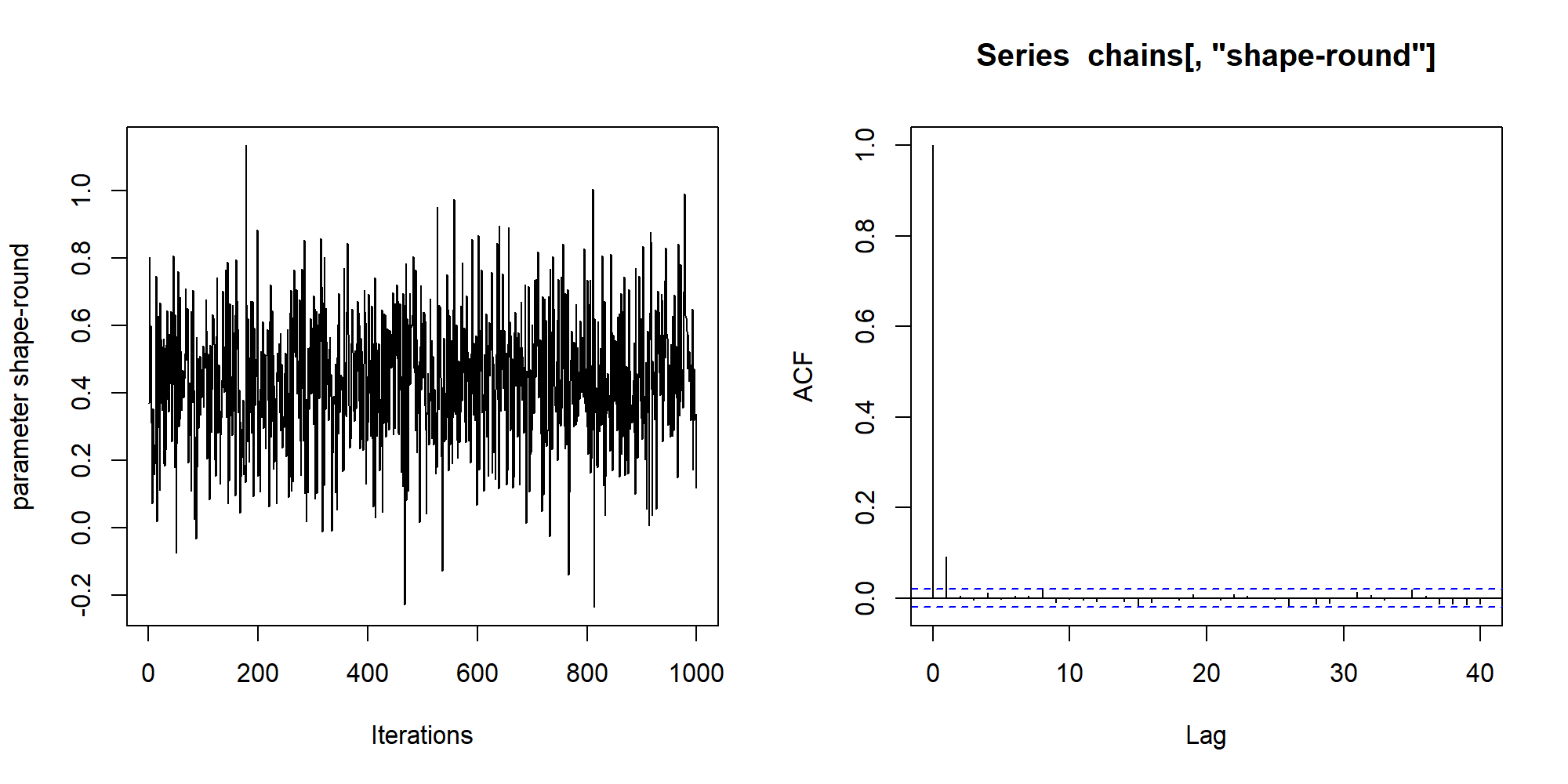The autocorrelation here is minimal, which will be the case in general for chains from the BayesFactor package. If we wanted to reduce it even further, we might consider thinning the chain: that is, keeping only every $$k$$ iterations. Thinning throws away information, and is generally not necessary or recommended; however, if memory is at a premium, we might prefer storing nearly independent samples to storing somewhat dependent samples.

The autocorrelation plot shows that the autocorrelation is reduced to 0 after 2 iterations. To get nearly independent samples, then, we could thin to every $$k=2$$ iterations using the thin argument:

chainsThinned = recompute(chains, iterations=20000, thin=2)

# check size of MCMC chain
dim(chainsThinned)

##  10000    26


Notice that we are left with 10,000 iterations, instead of the 20,000 we sampled, because half were thinned. The figure below shows the resulting MCMC chain and autocorrelation functions. The MCMC chain does not visually look very different, because the autocorrelation was minimal in the first place. However, the autocorrelation function no longer shows autocorrelation from one iteration to the next, implying that we have obtained 10,000 nearly independent samples.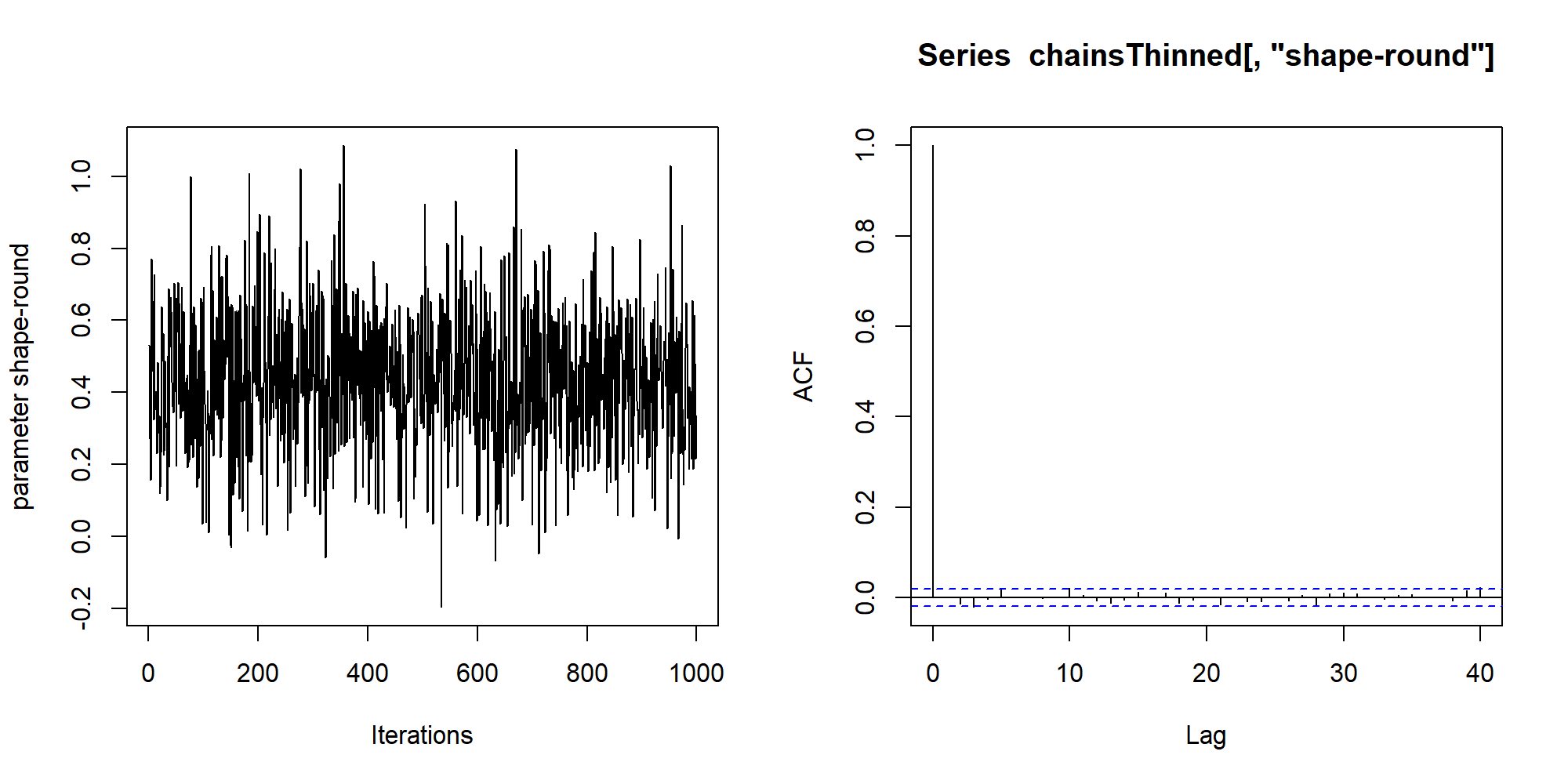### Fine-tuning of prior scales (0.9.12-2+)

Previous to version 0.9.12-2, it was only possible to change the priors on a per-effect-type basis; ie, fixed effects all had the same prior scale, random effects had a different prior scale, and slopes had third prior scale. As of 0.9.12-2, it is possible to change the prior on a per-effect basis for fixed and random effects (slopes still share a common prior, due to the use of the Liang et al. hyper-g priors for the slopes). This is accomplished via the rscaleEffects argument to lmBF, anovaBF, and generalTestBF.

The rscaleEffects argument is a named vector. The names correspond to the effect you'd for which you'd like to set the prior, and the value is the prior scale value. Any settings in rscaleEffects will override the settings in rscaleFixed and rscaleRandom; if no settings are found in rscaleEffects, then the settings in rscaleFixed and rscaleRandom are used.

We can demonstrate using the puzzles data set. Suppose we prefer a prior on the color main effect of $$r=1$$, a prior twice as wide as the default in rscaleFixed, $$r=.5$$. We set the prior scale for color using the rscaleEffects argument:

newprior.bf = anovaBF(RT ~ shape + color + shape:color + ID, data = puzzles,
whichRandom = "ID",rscaleEffects = c( color = 1 ))

newprior.bf

## Bayes factor analysis
## --------------
##  shape + ID                       : 2.8  ±0.75%
##  color + ID                       : 2.14 ±1.74%
##  shape + color + ID               : 8.97 ±1.66%
##  shape + color + shape:color + ID : 3.36 ±2.18%
##
## Against denominator:
##   RT ~ ID
## ---
## Bayes factor type: BFlinearModel, JZS


The other fixed effects, shape and shape:color, retain the prior scale of $$r=.5$$ from rscaleFixed. Compare these Bayes factors to the ones with in the mixed modeling section above.